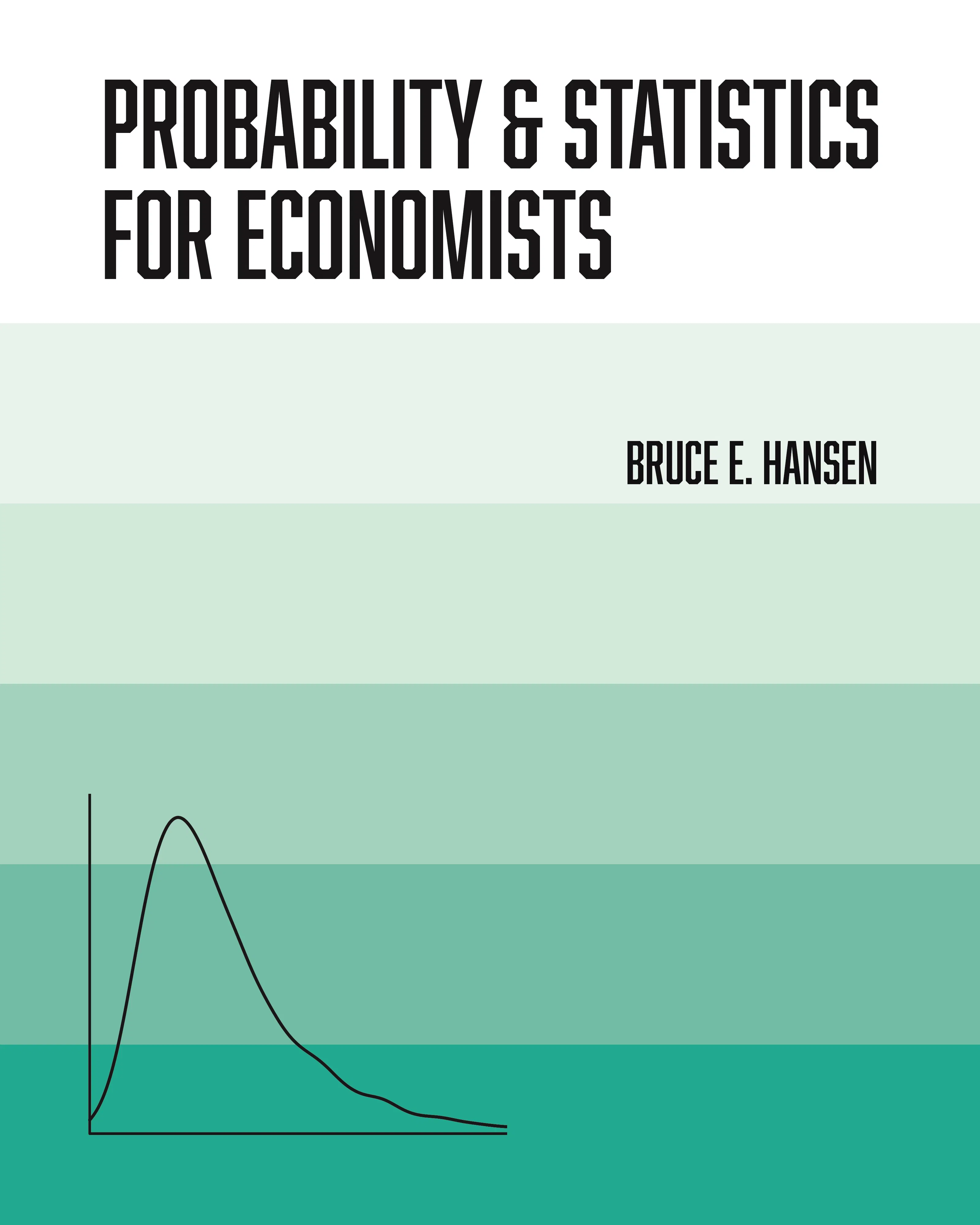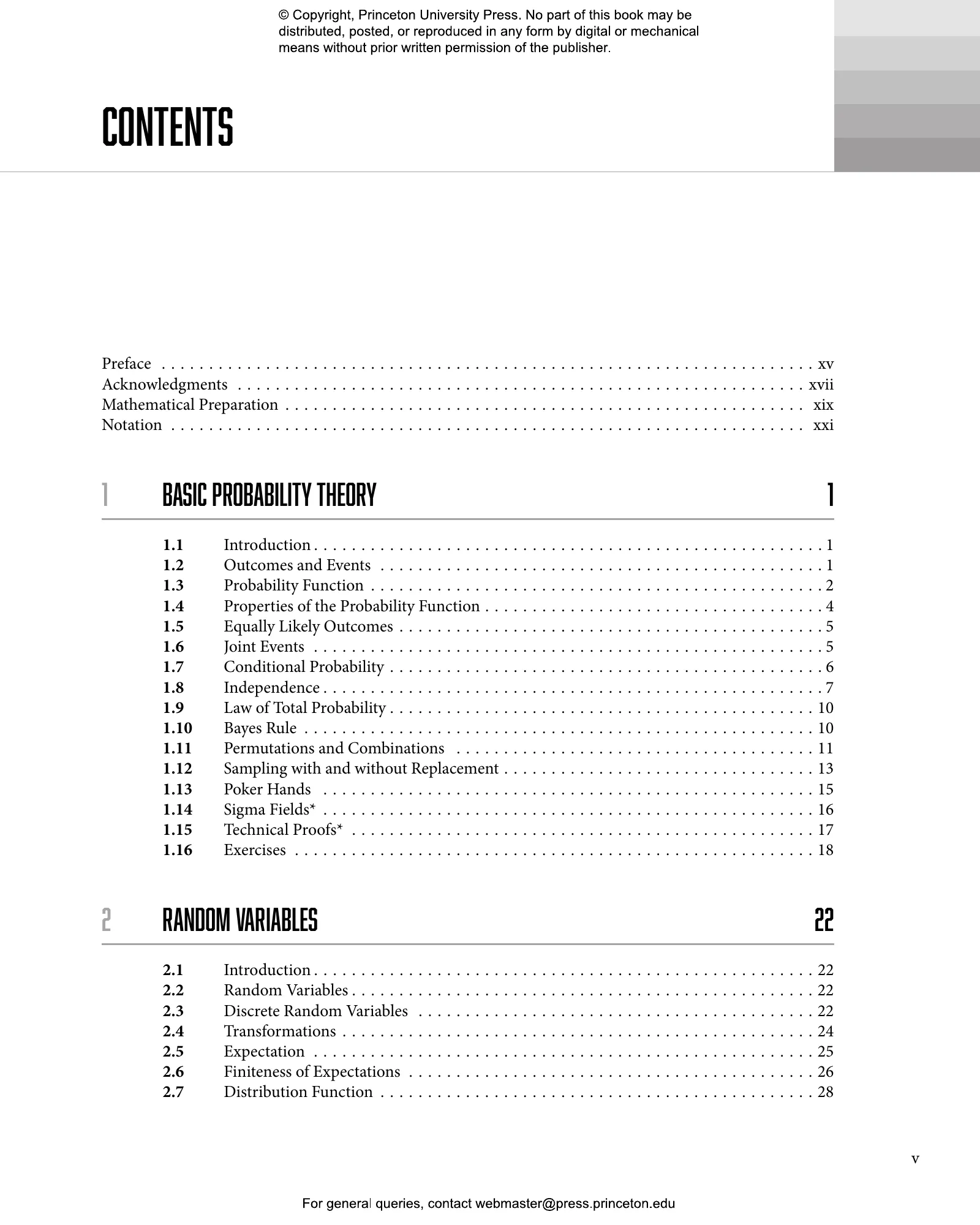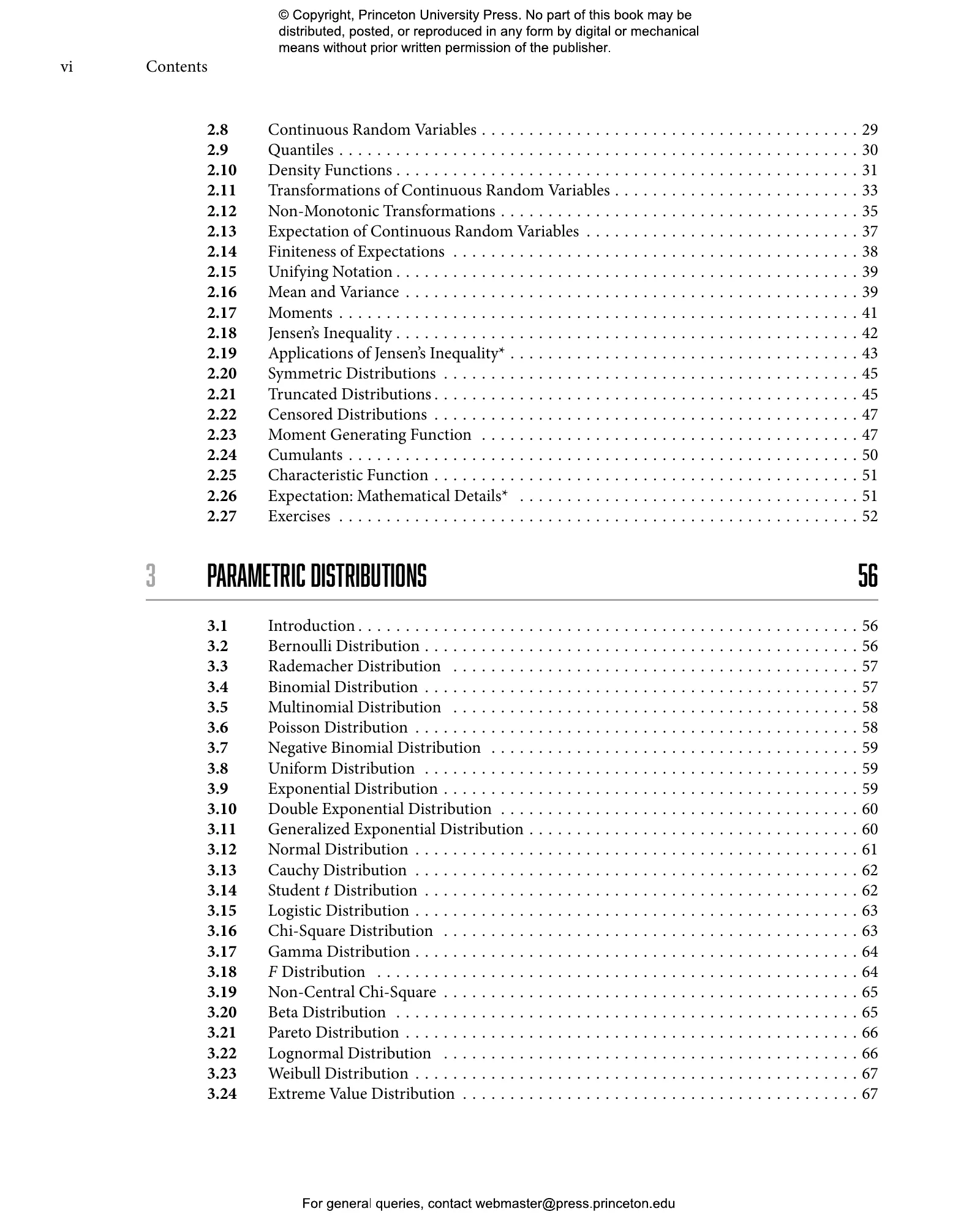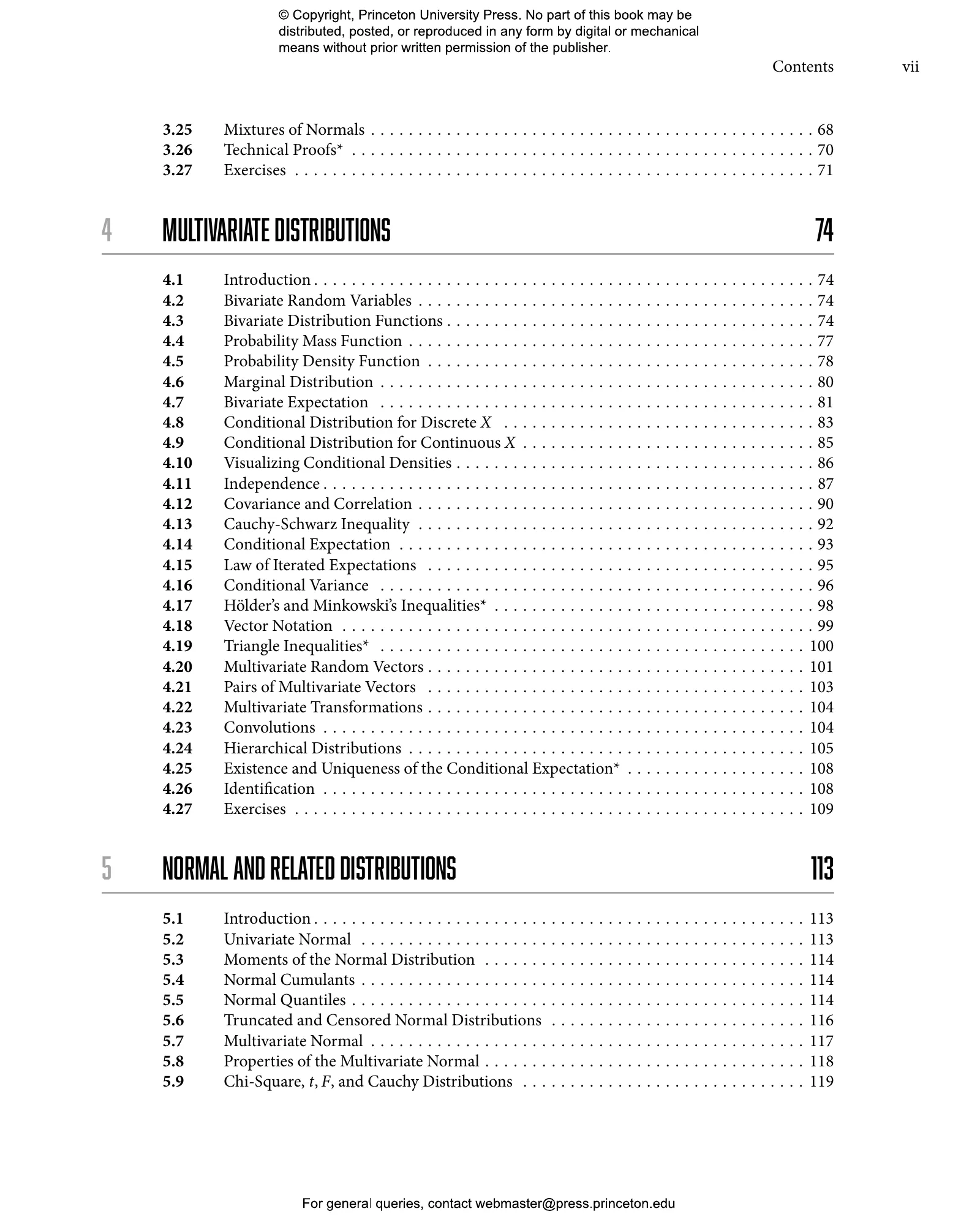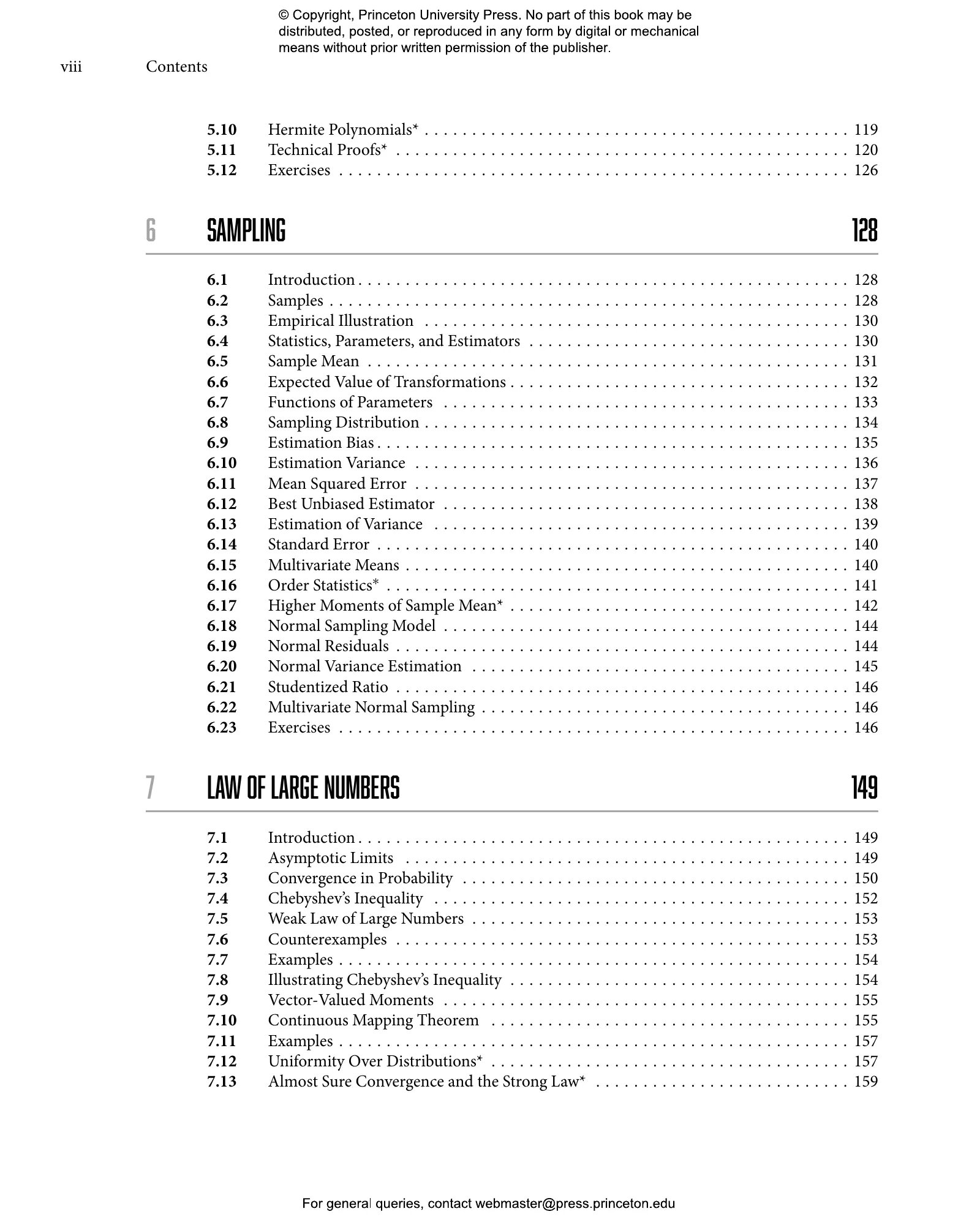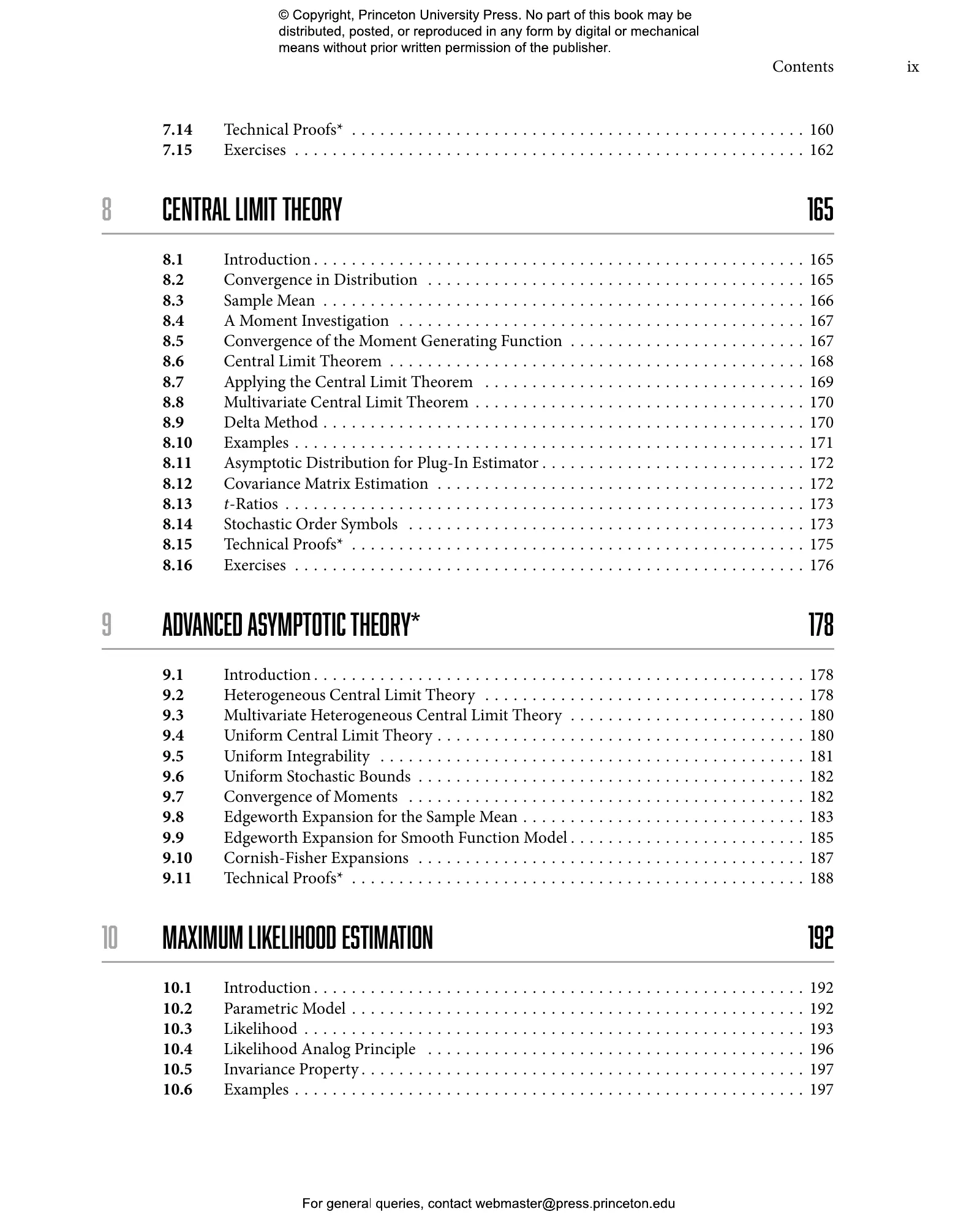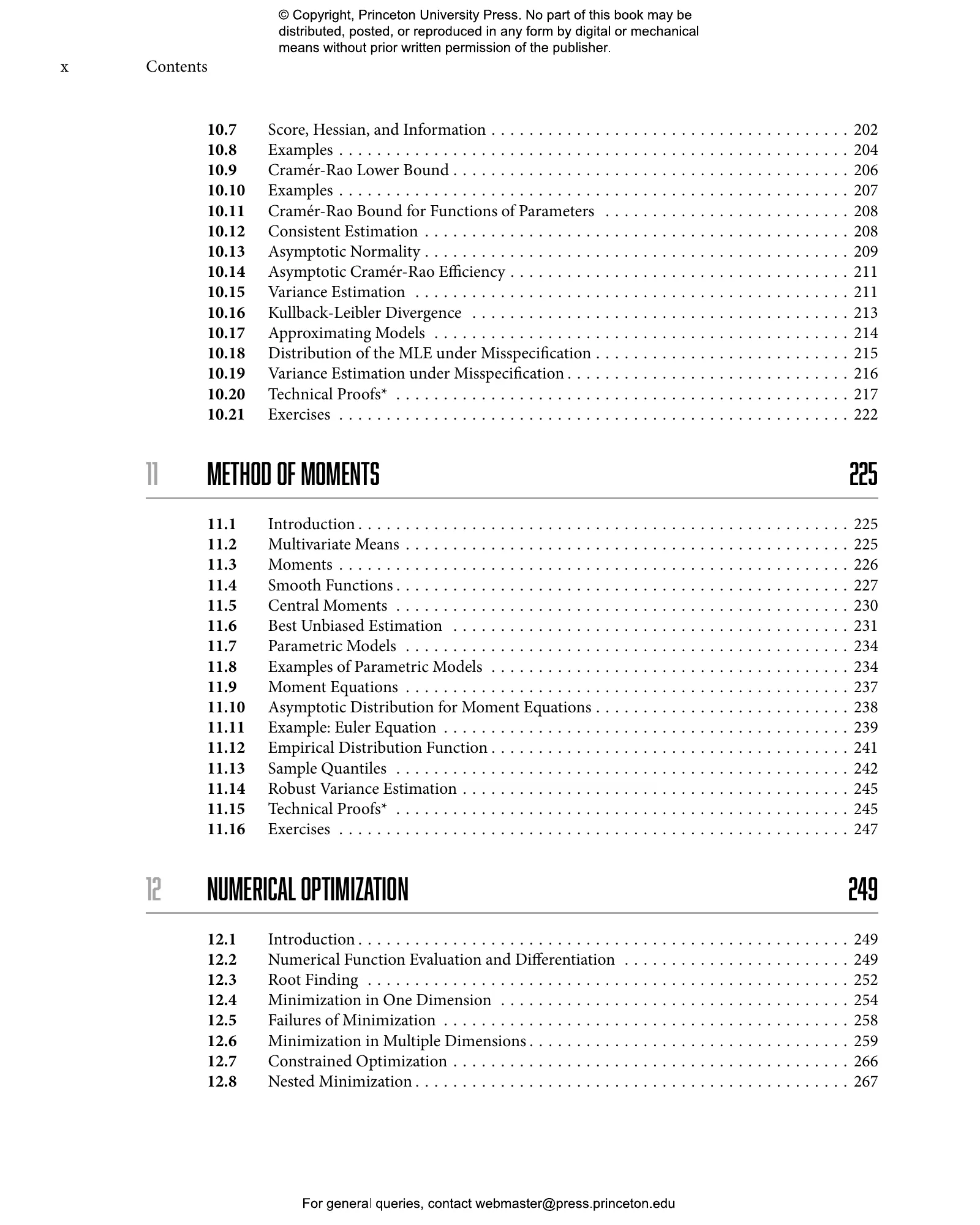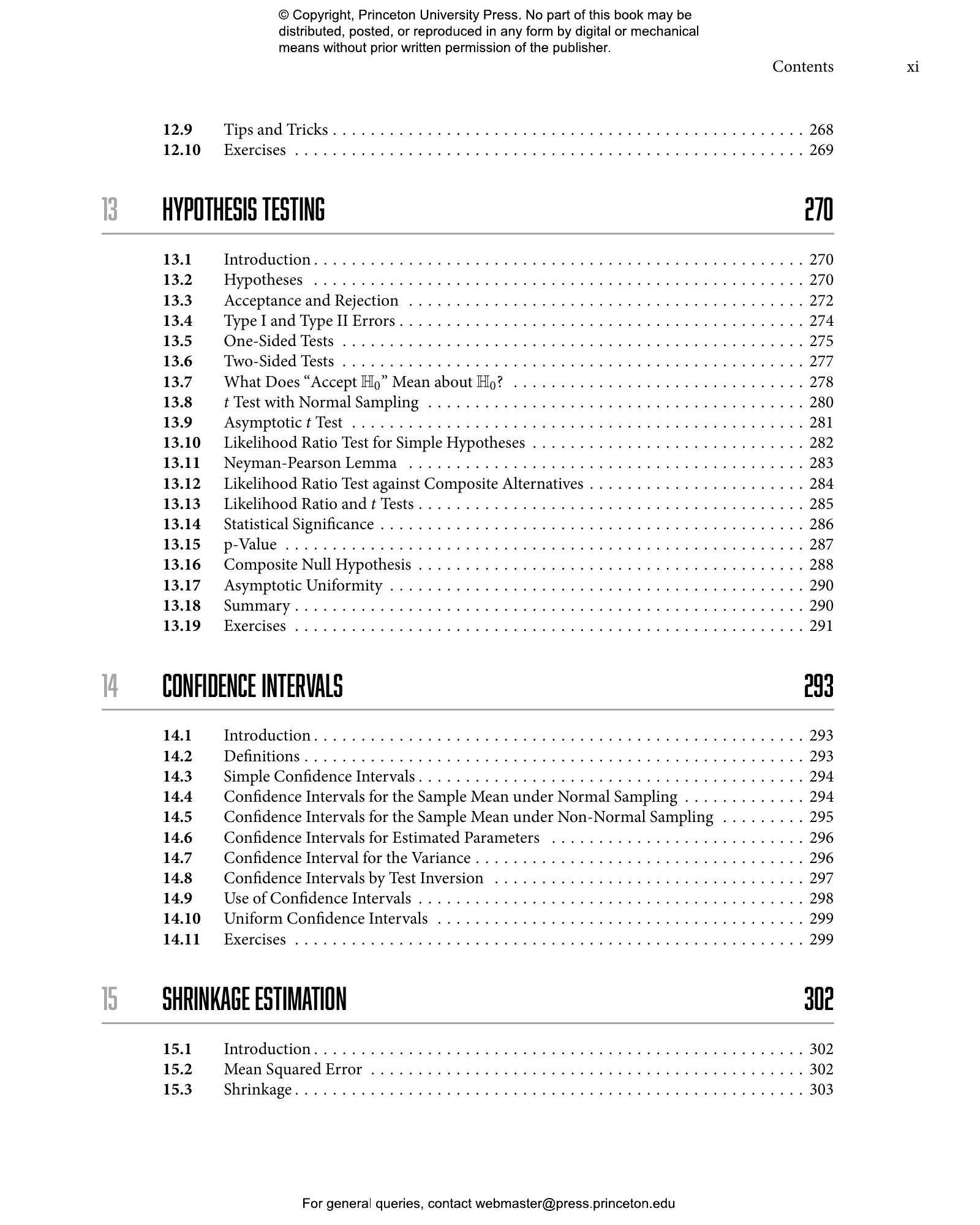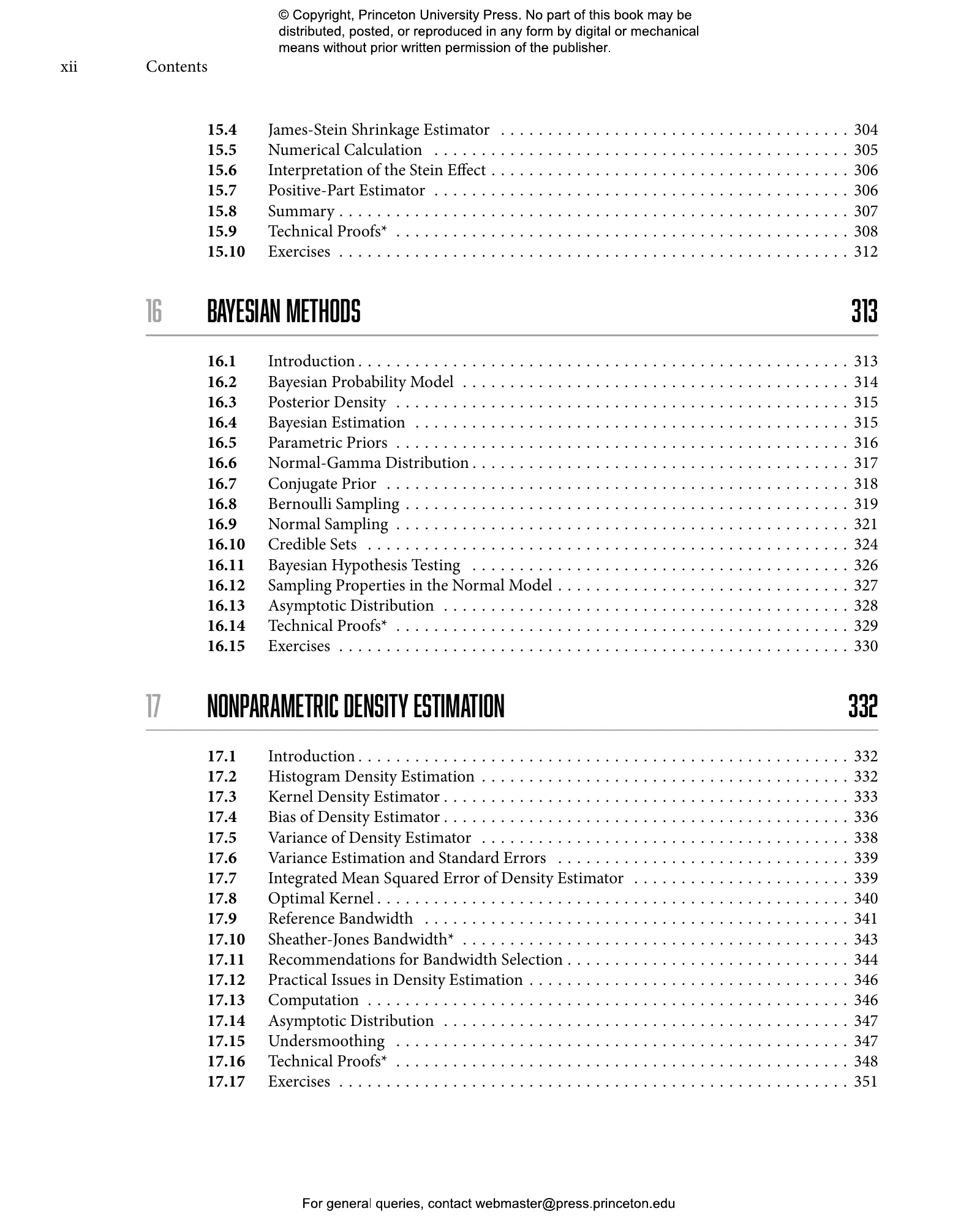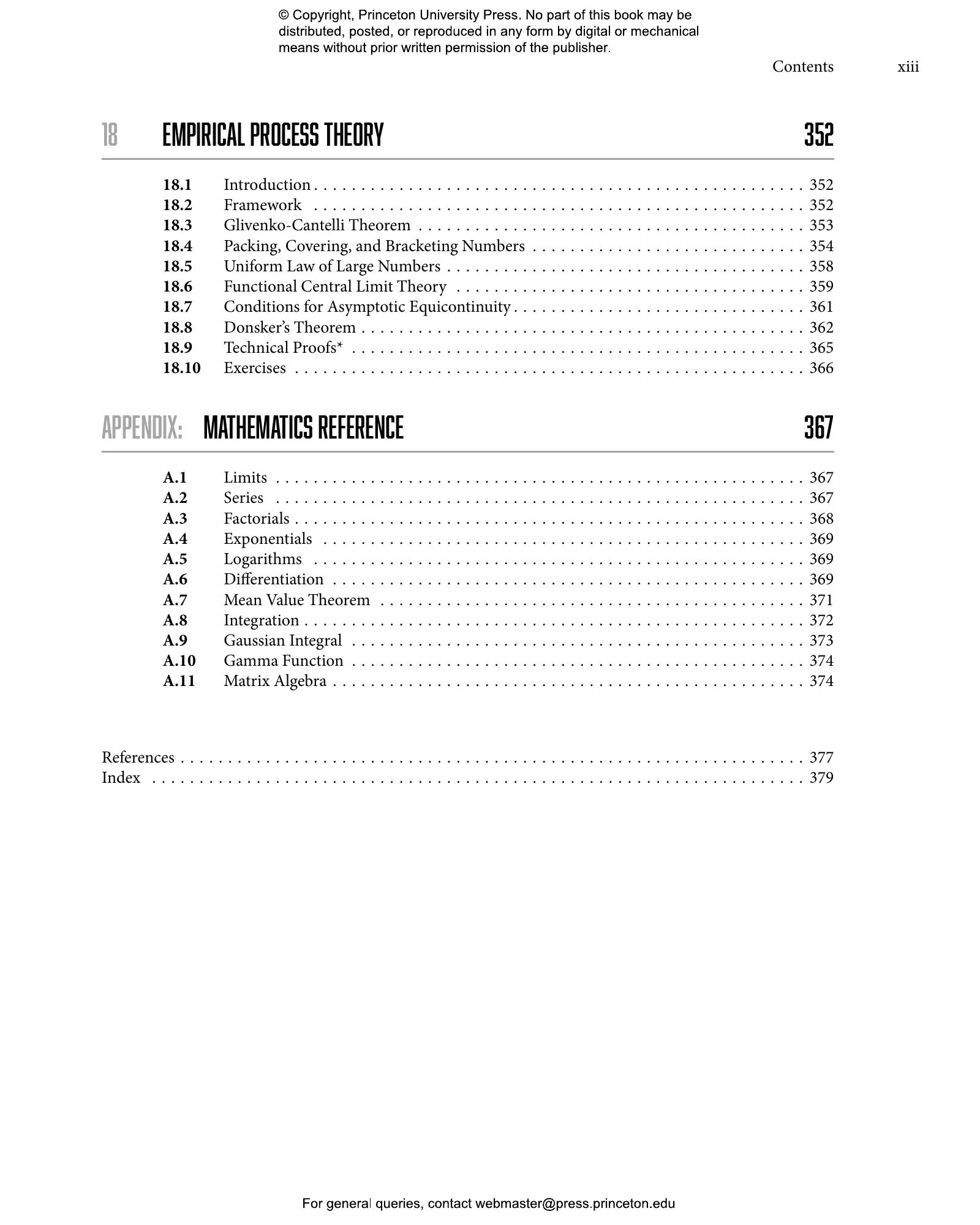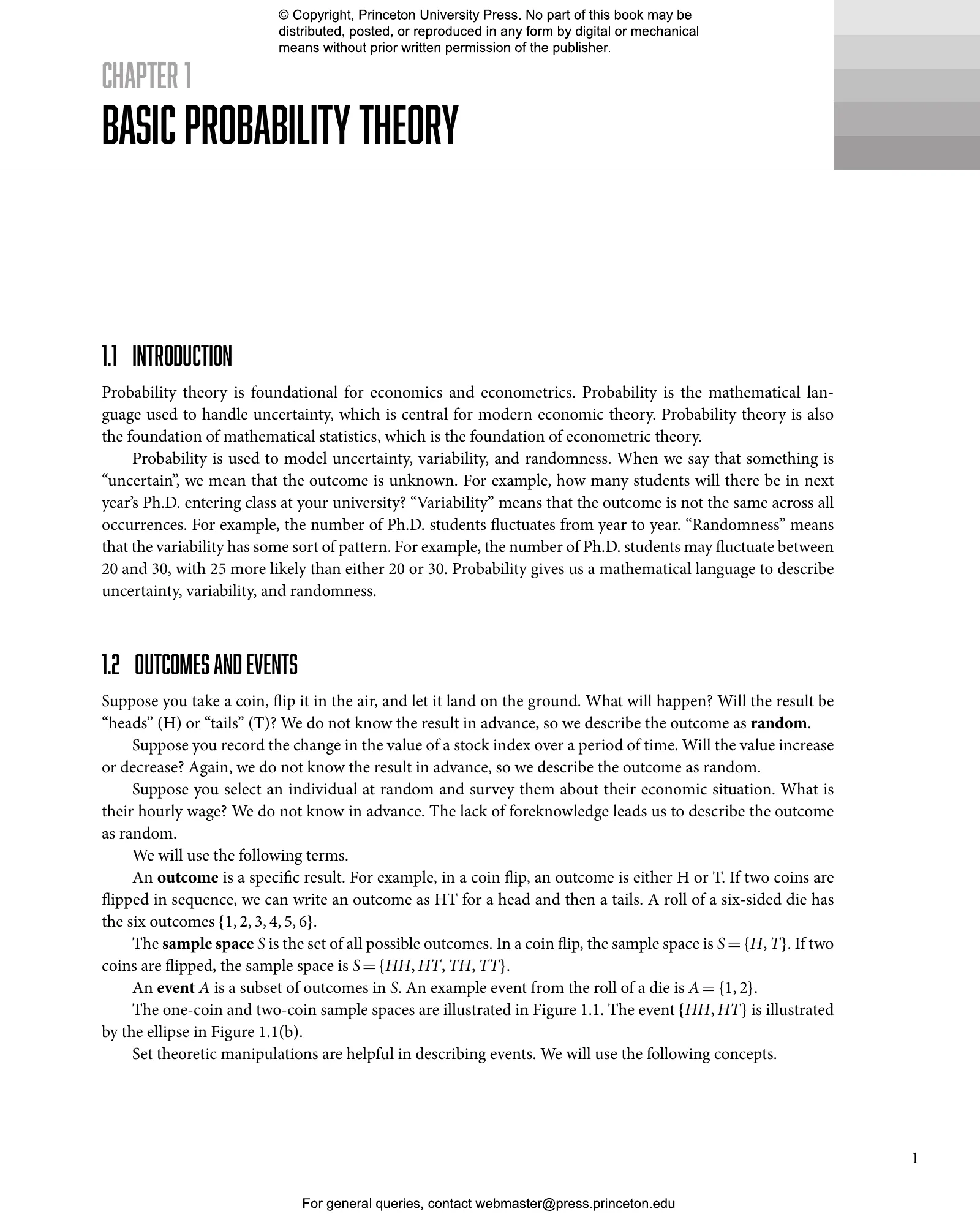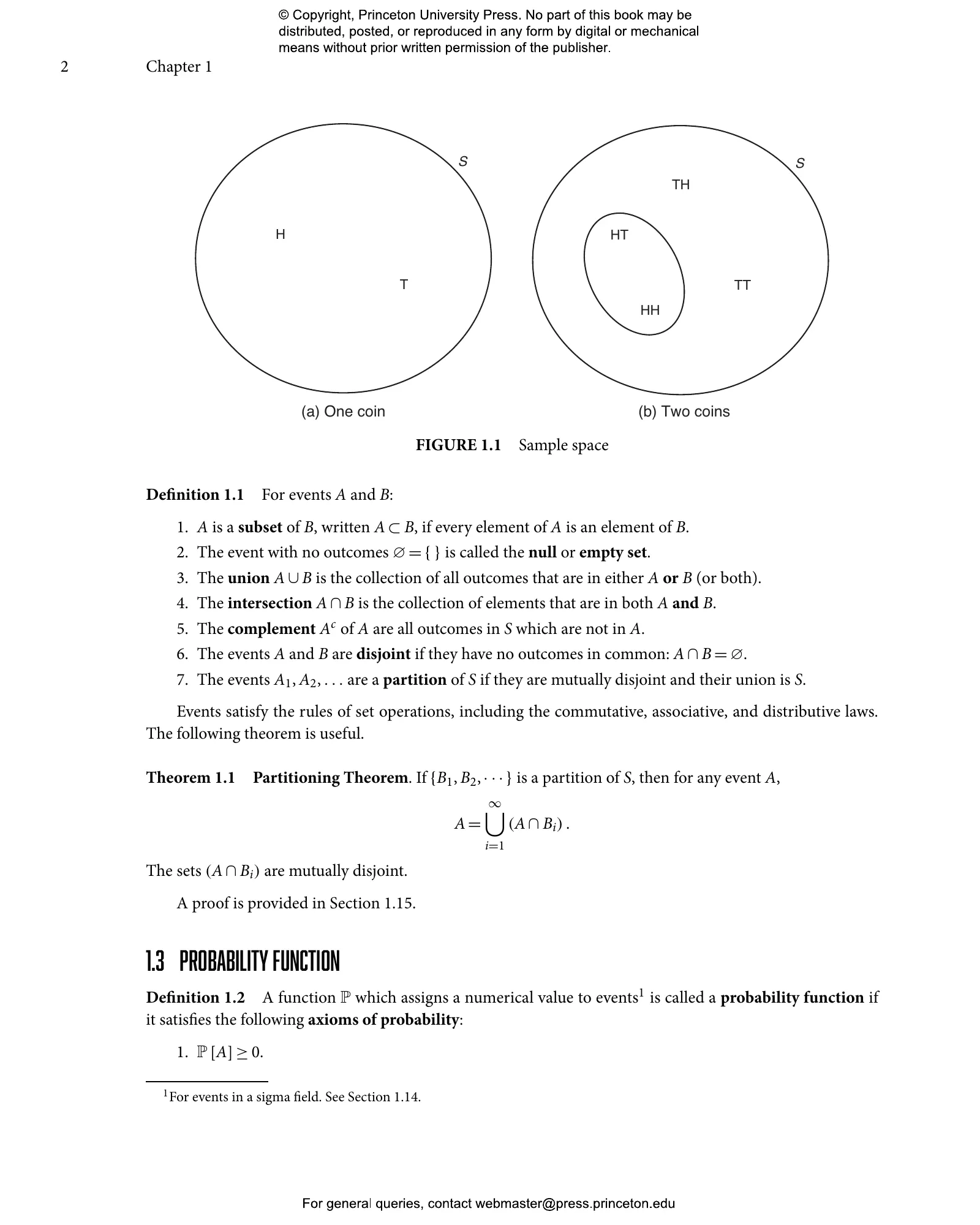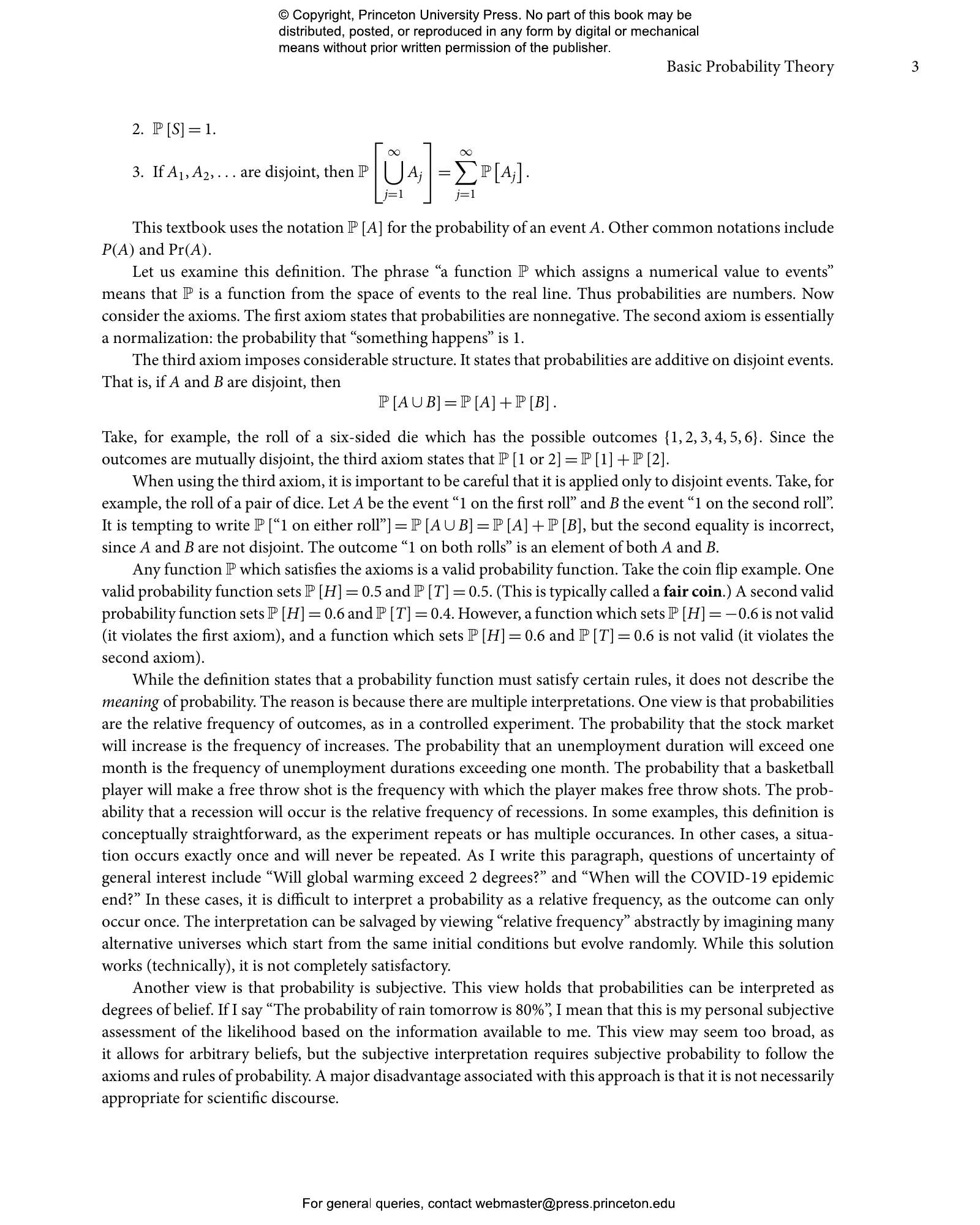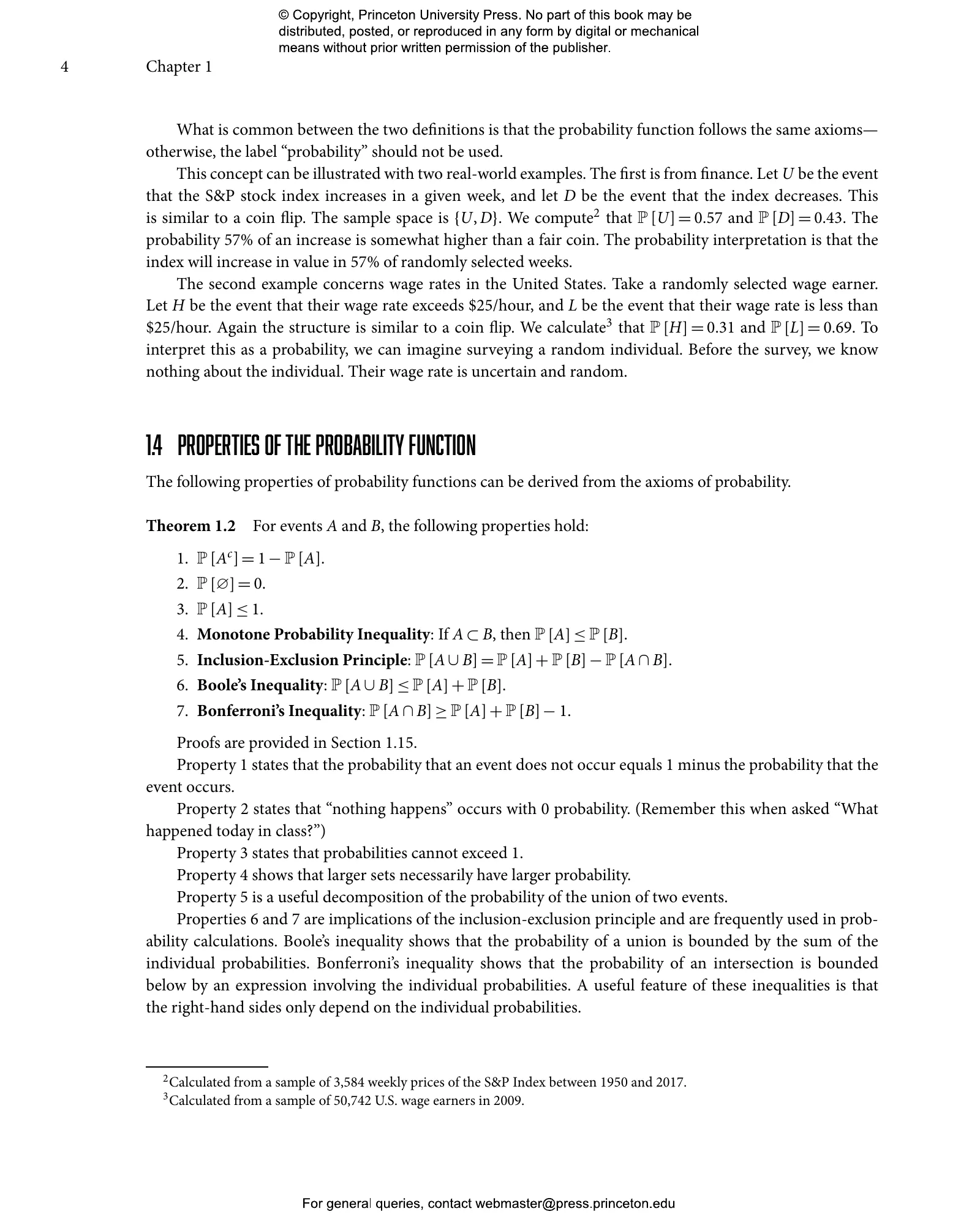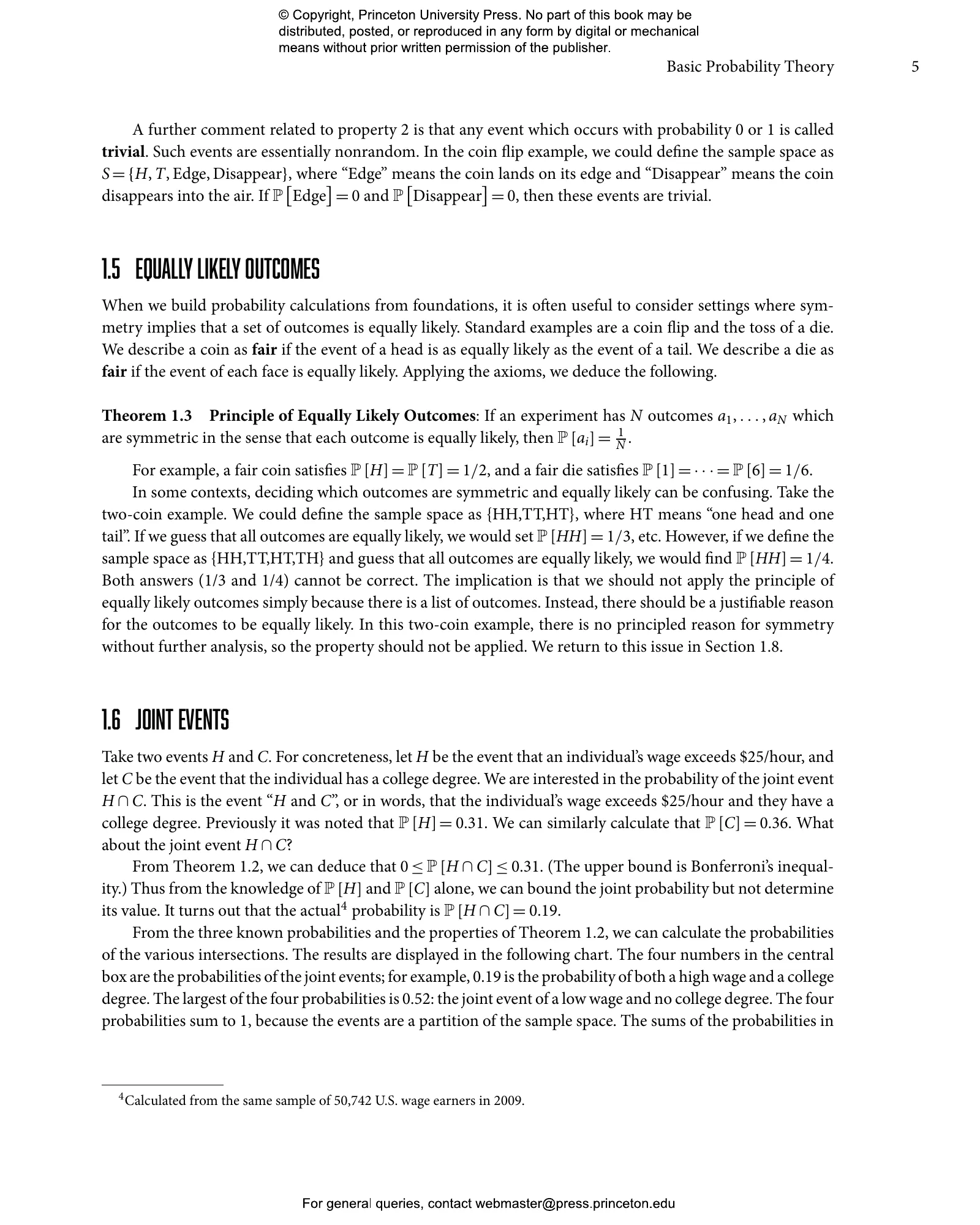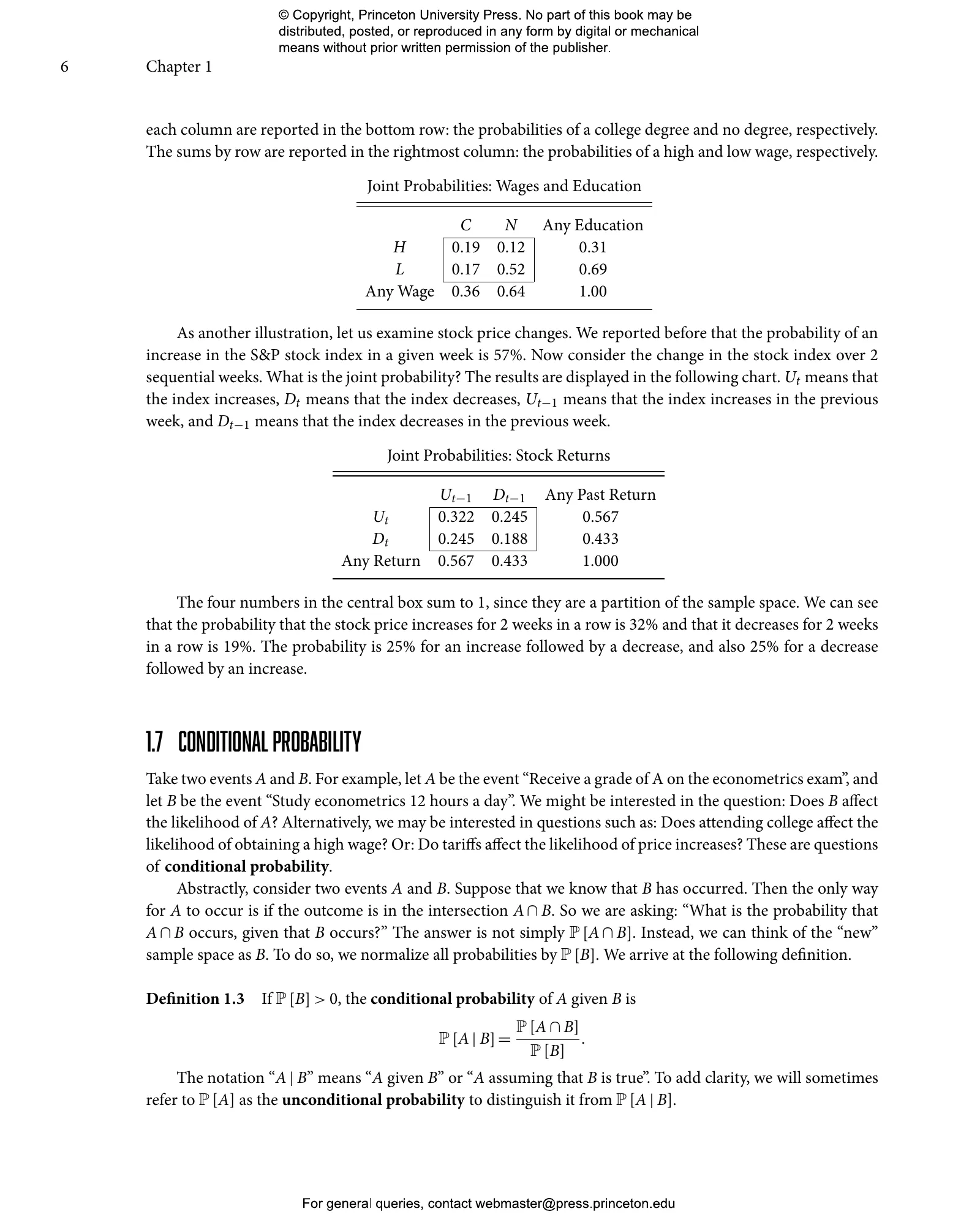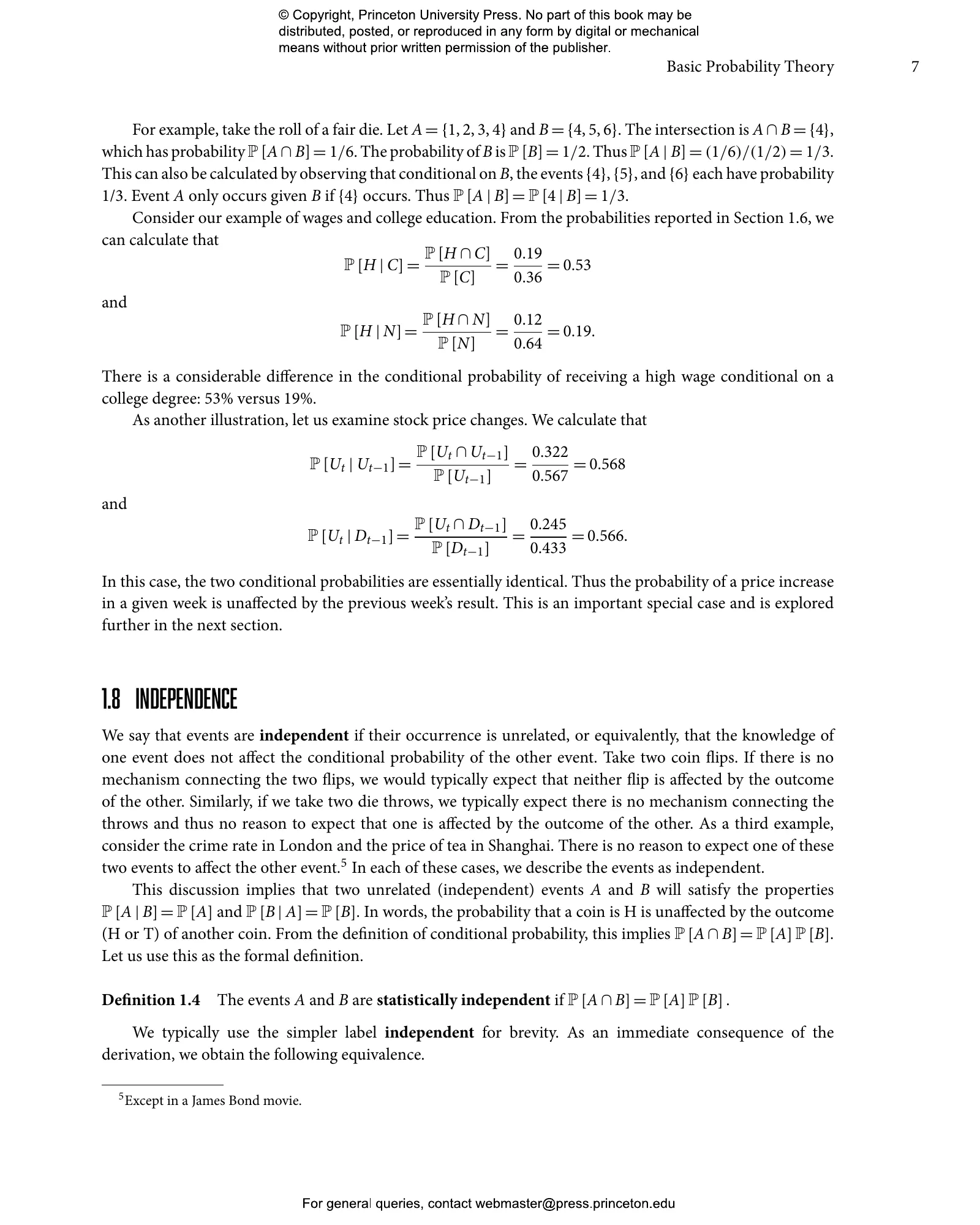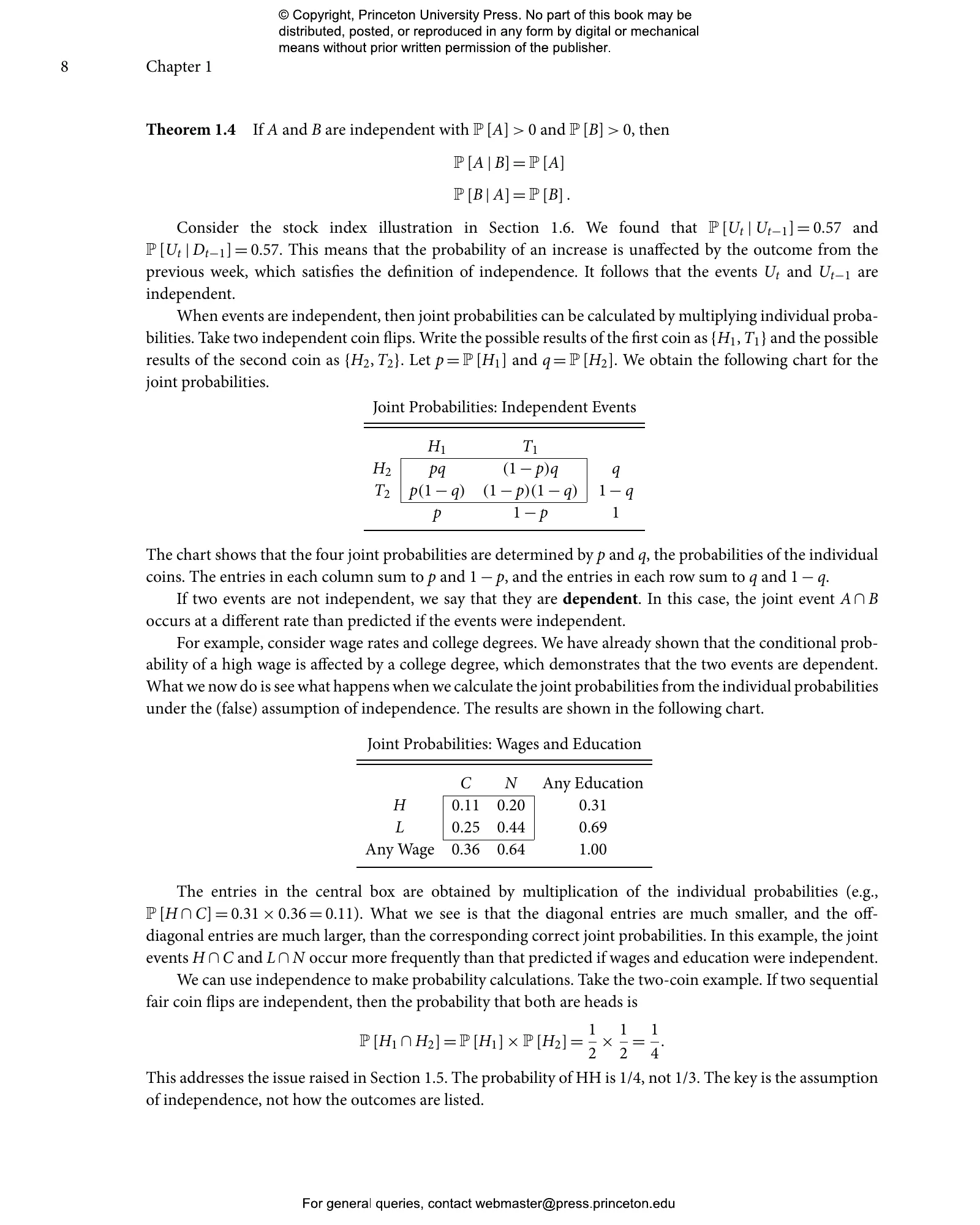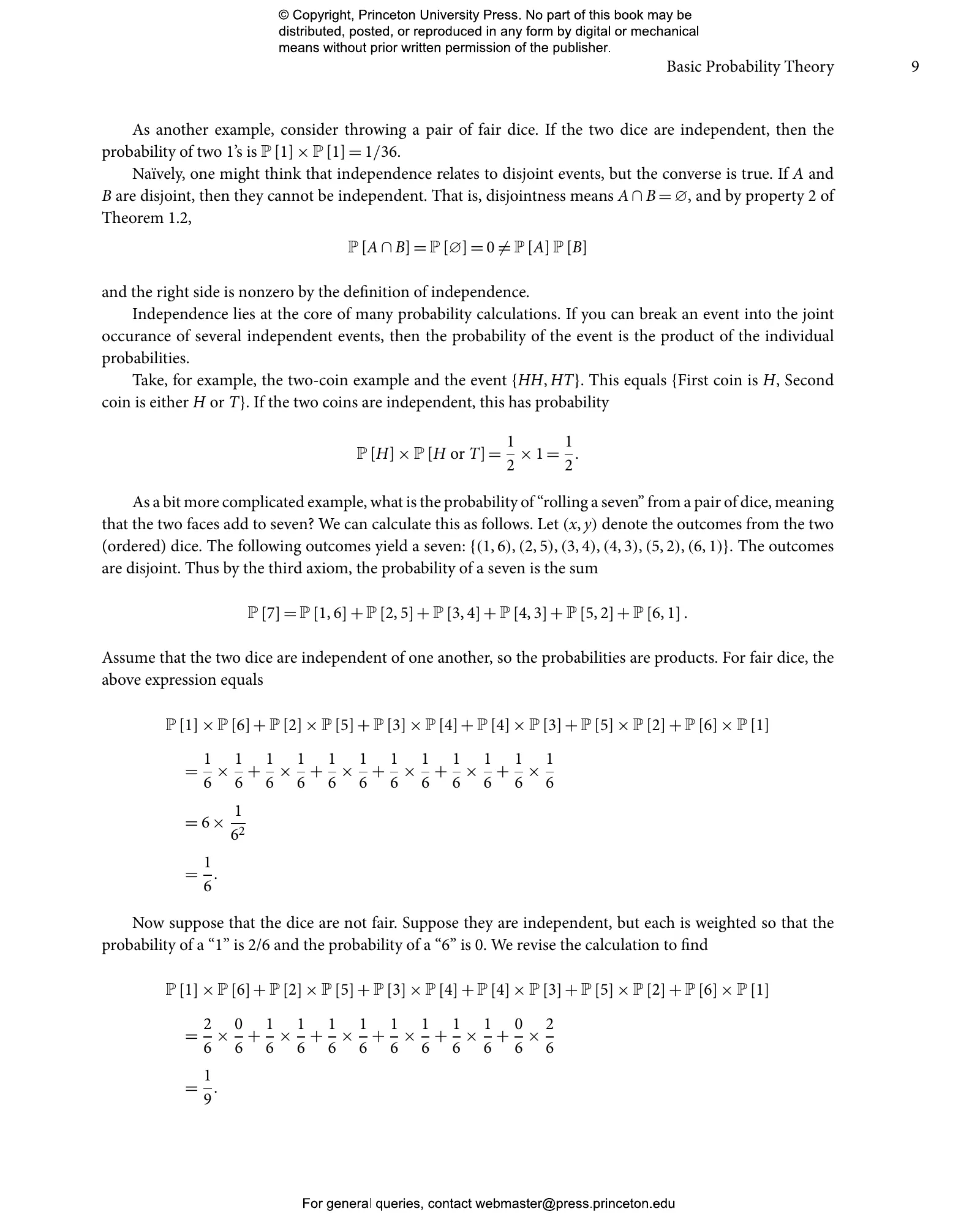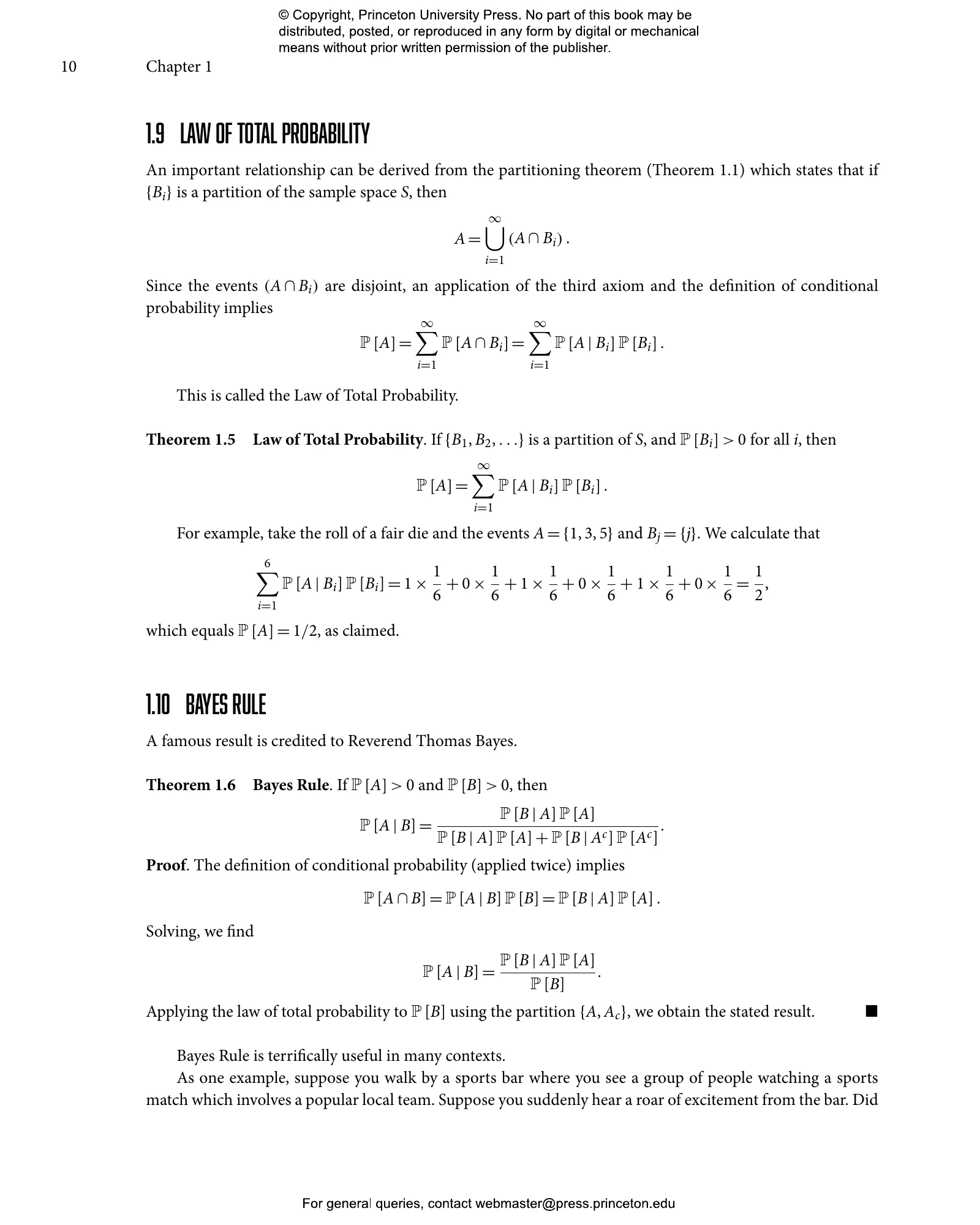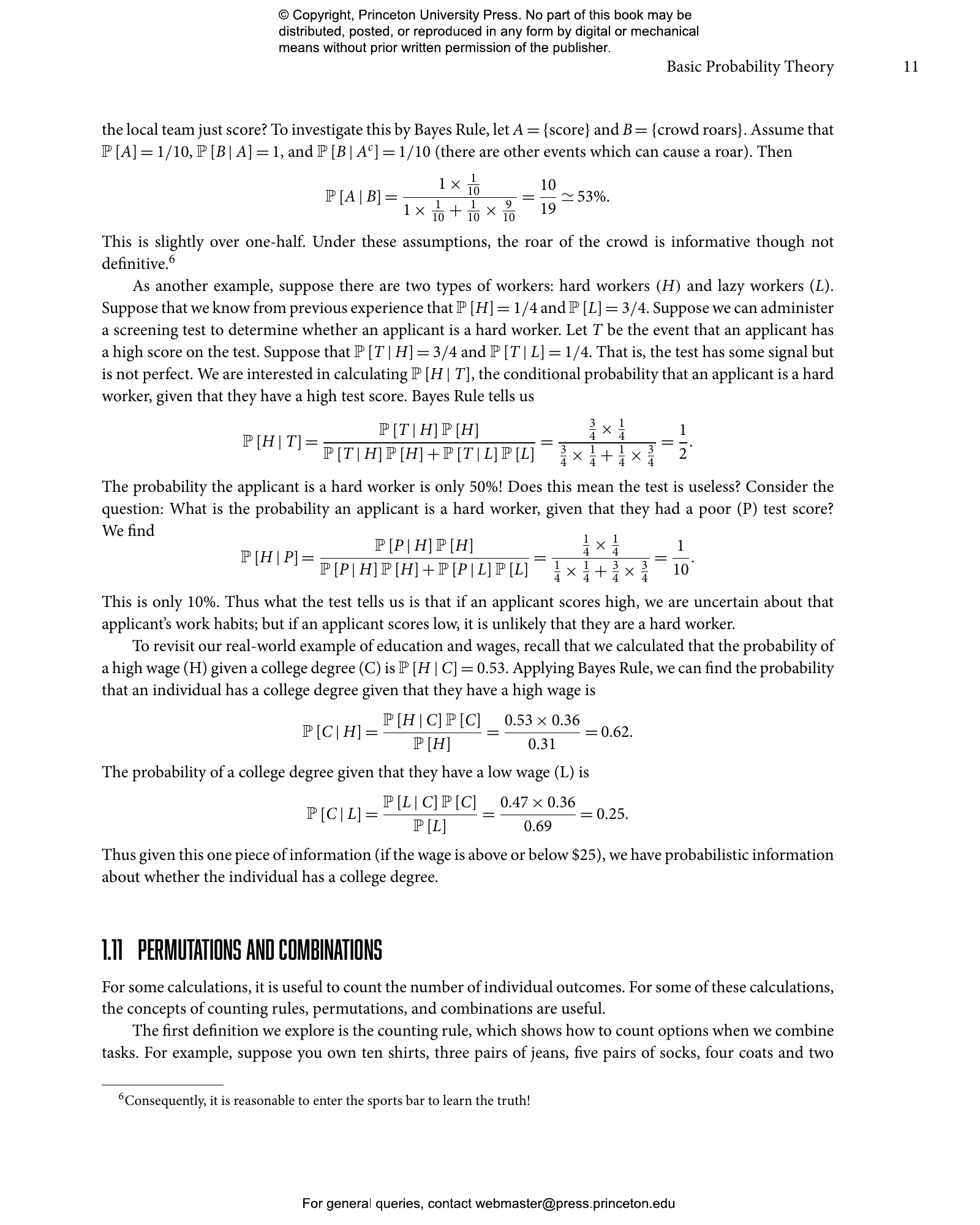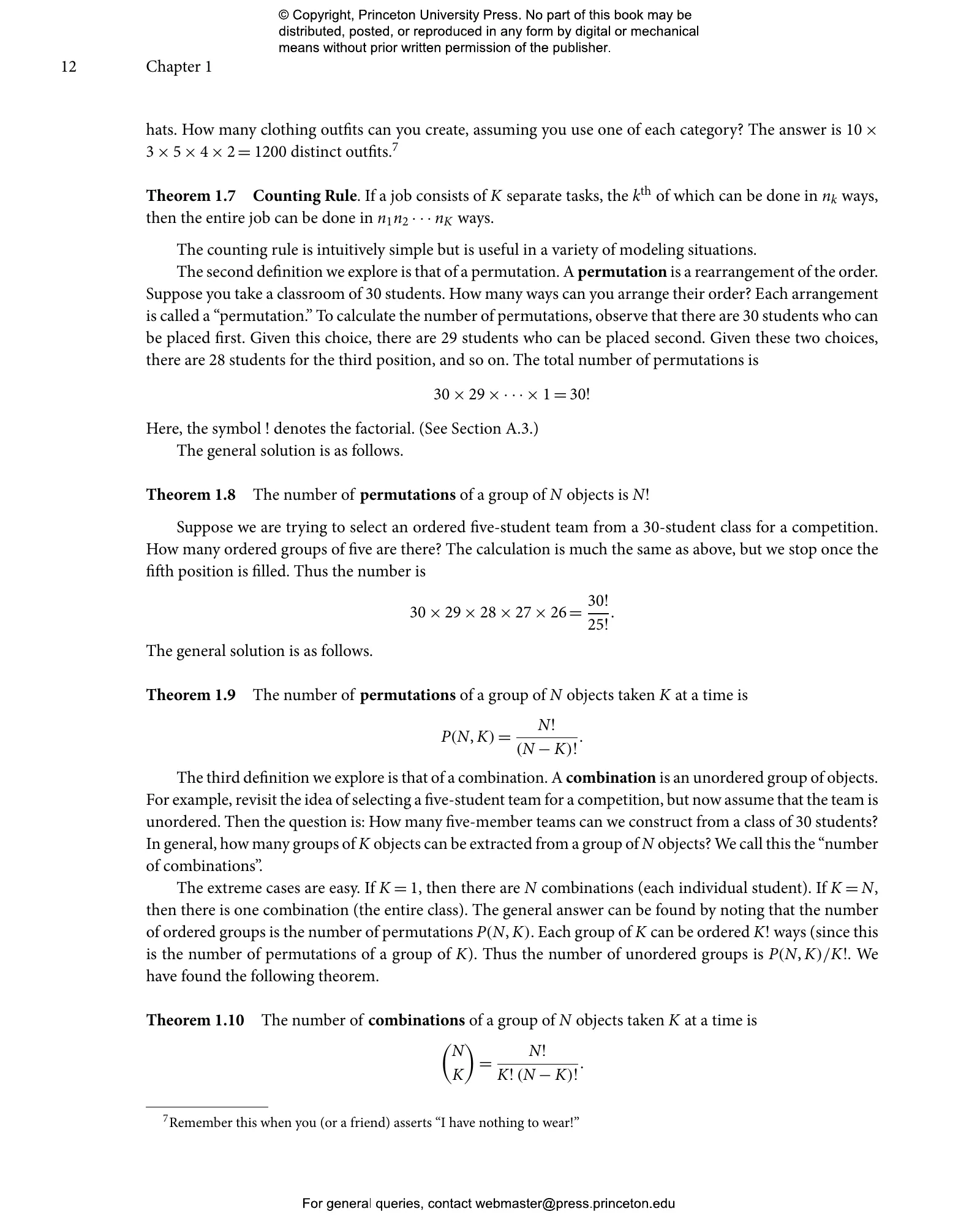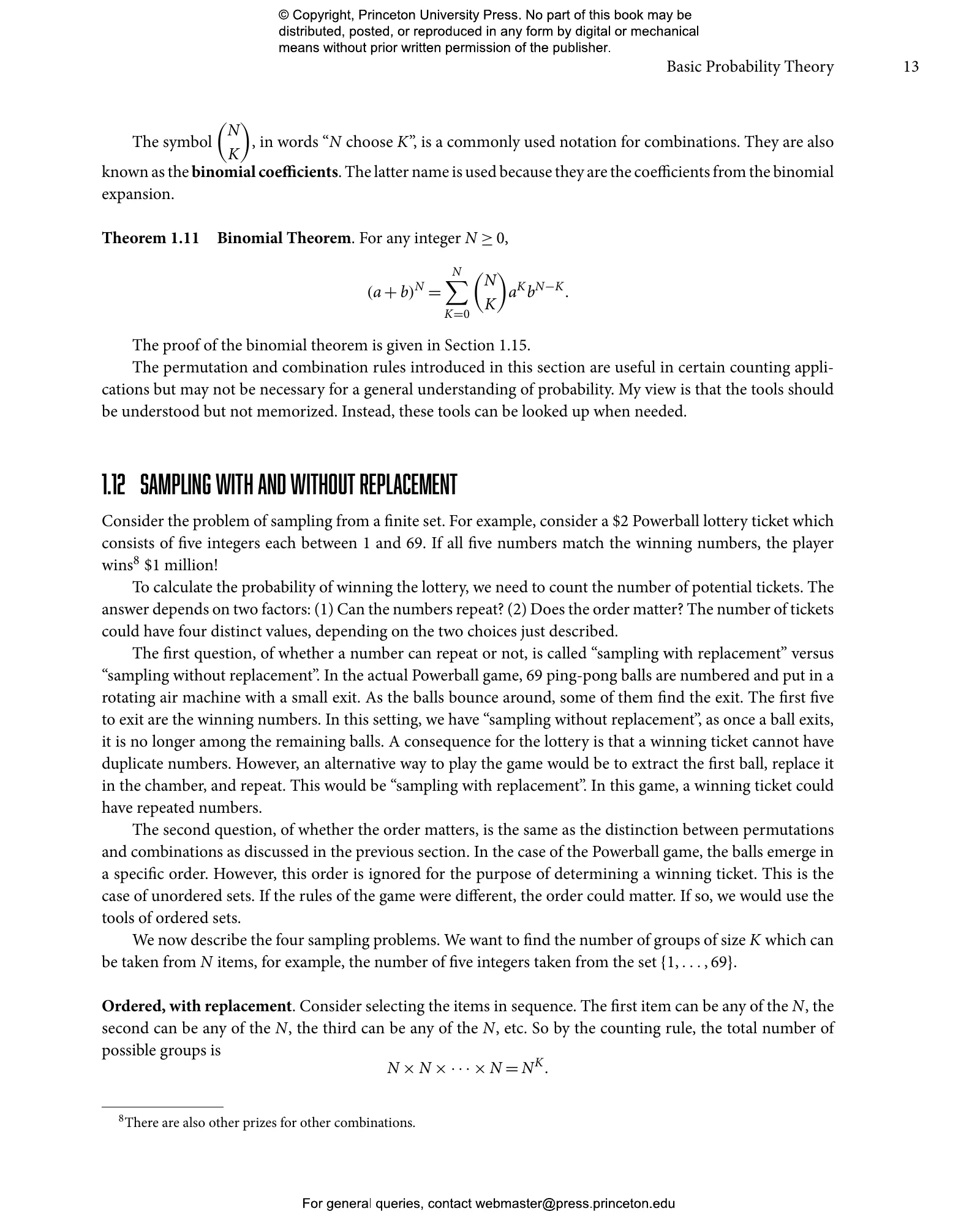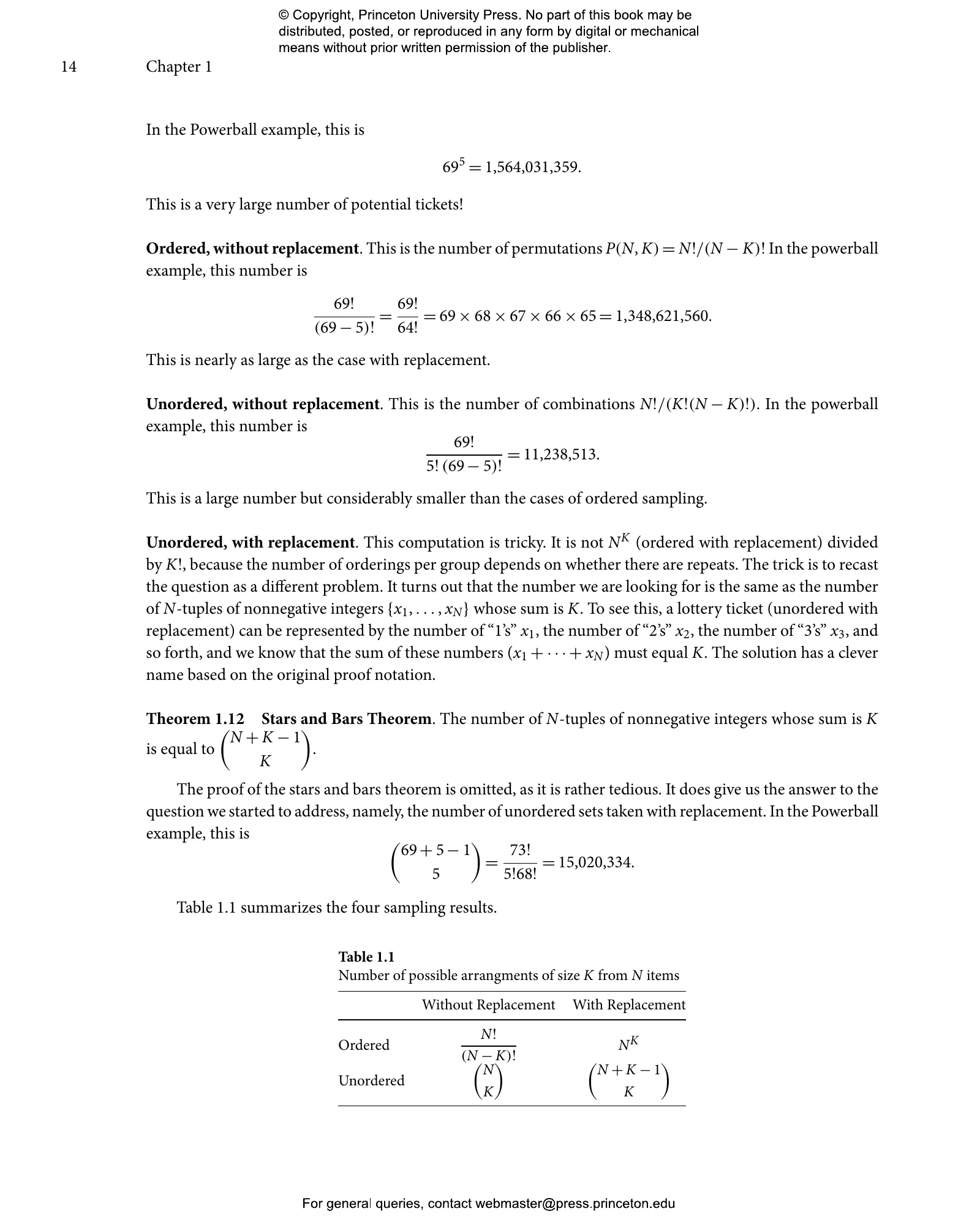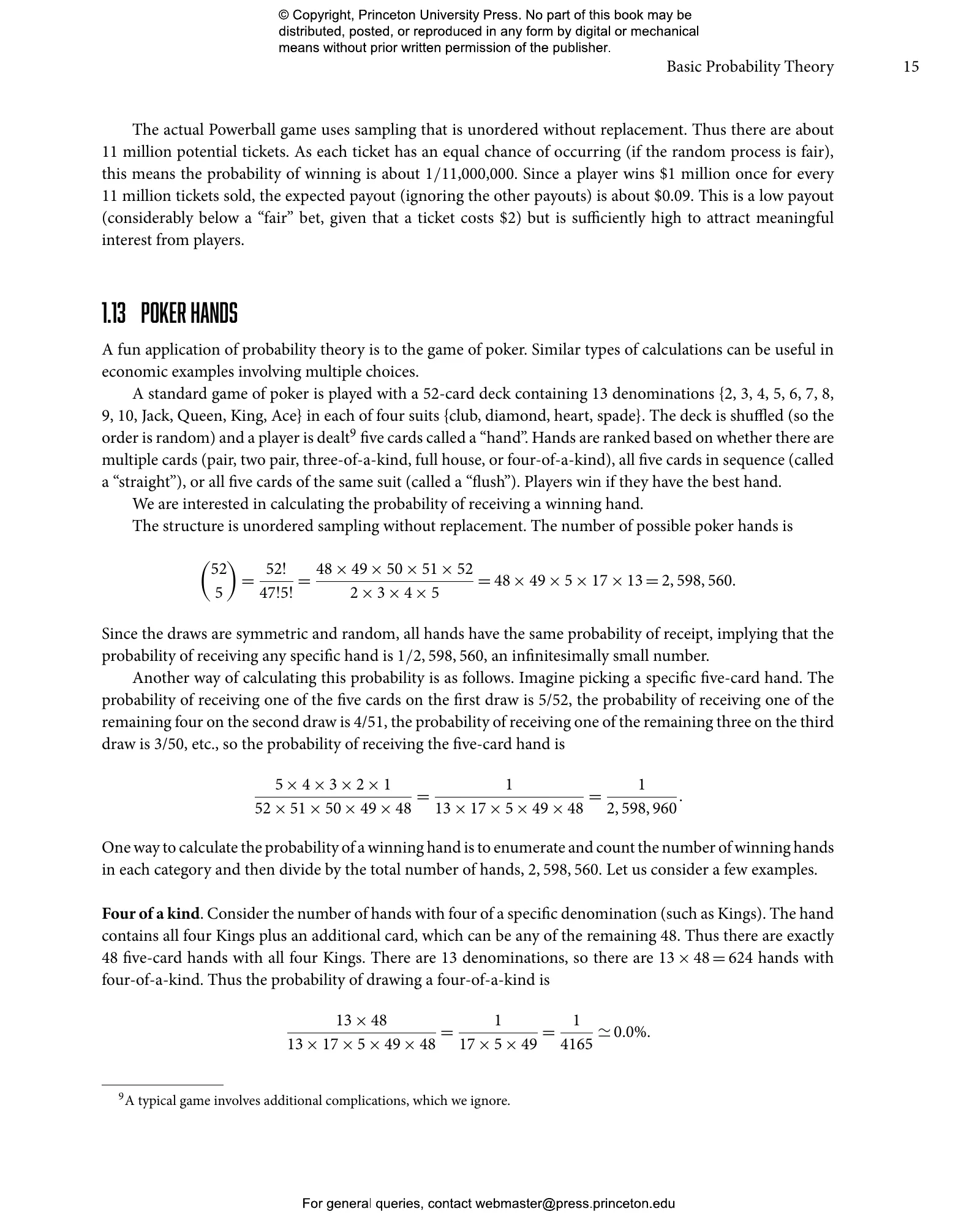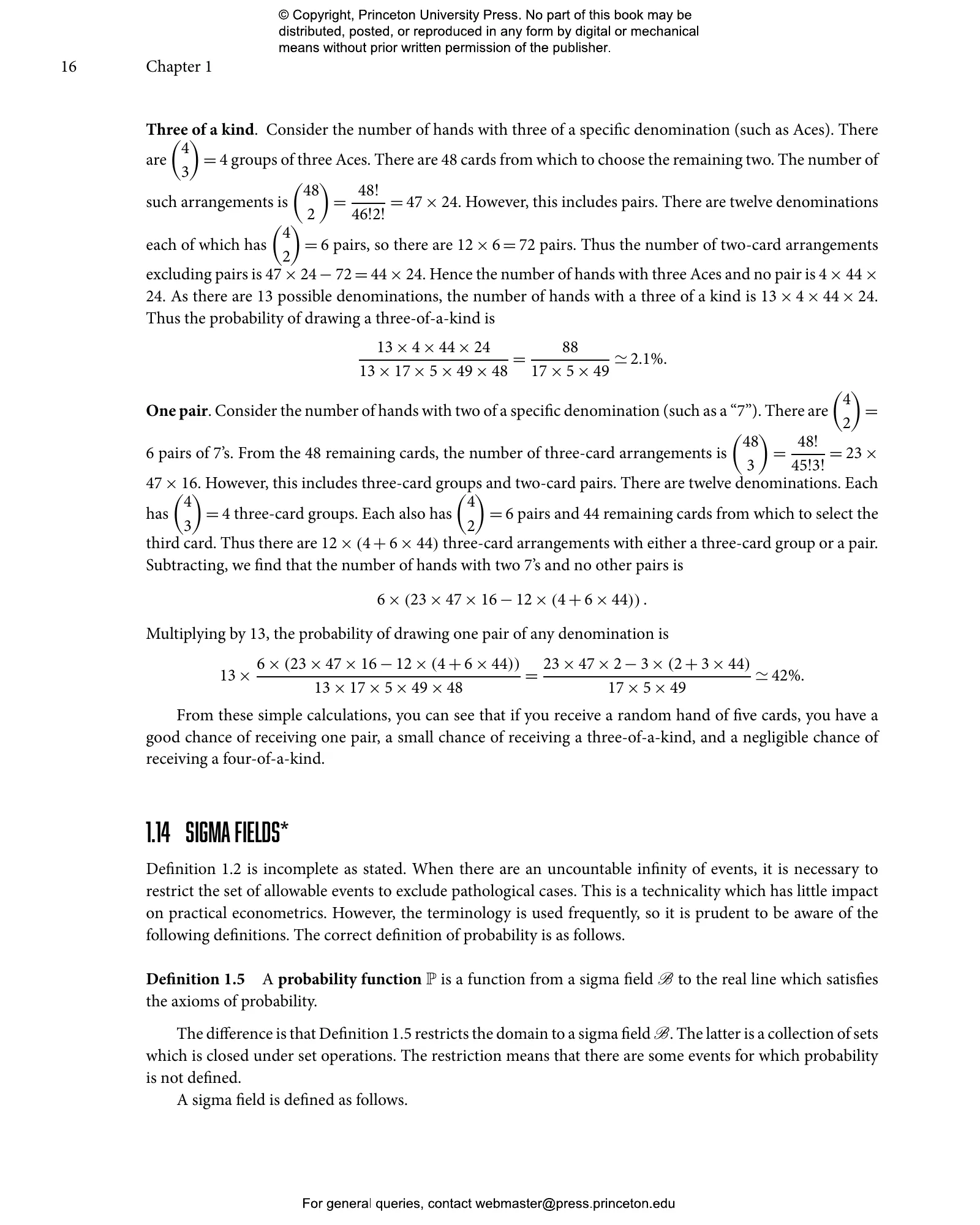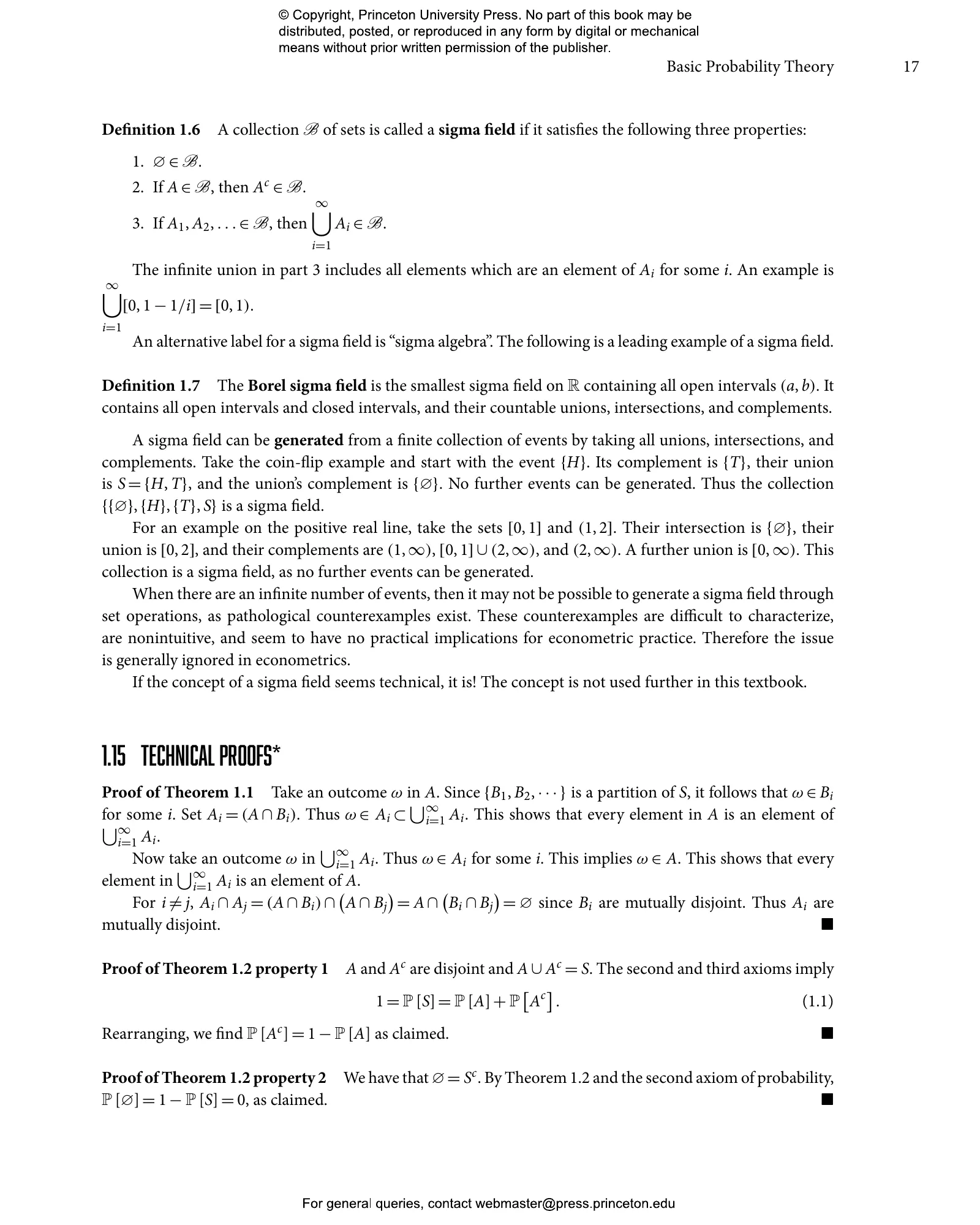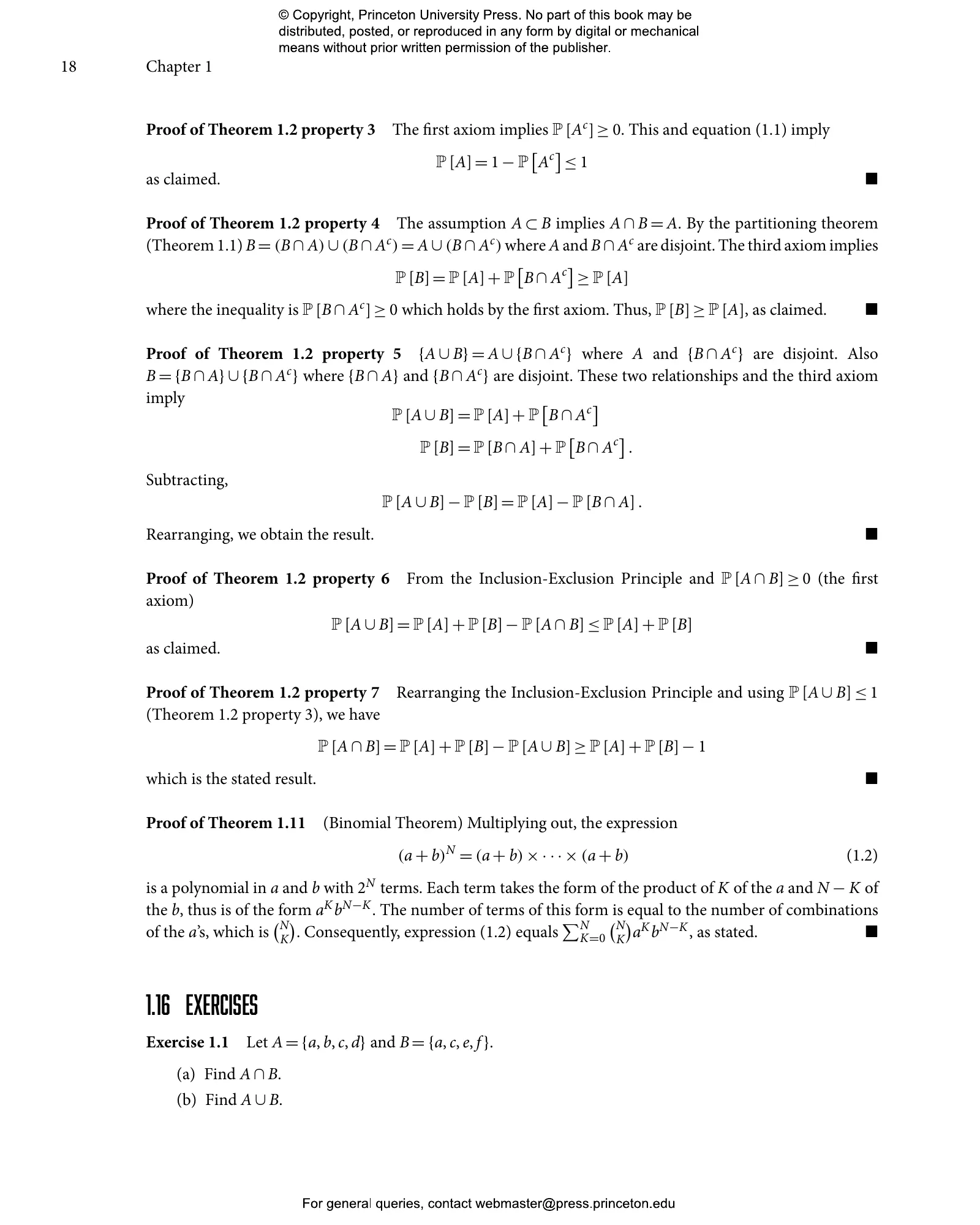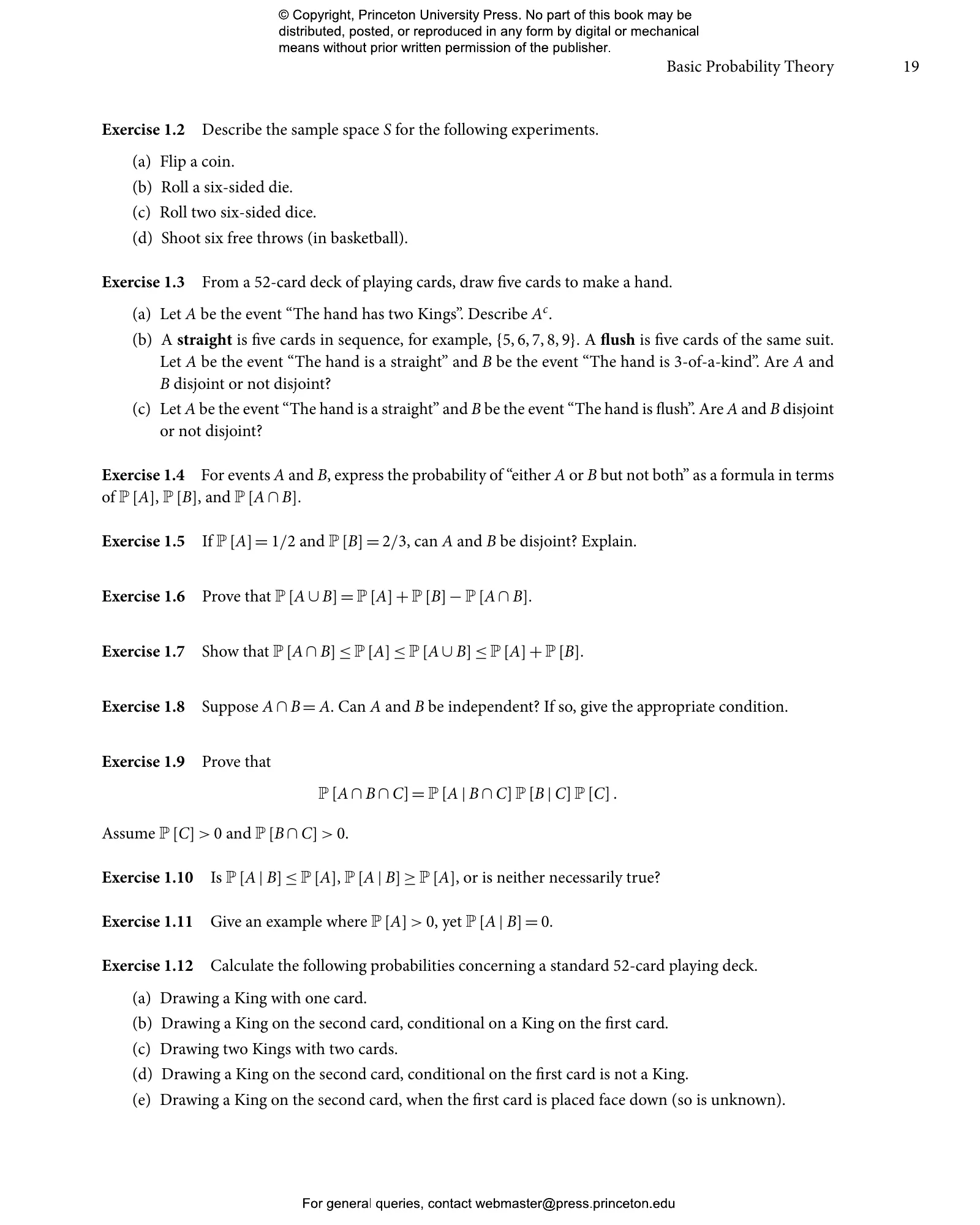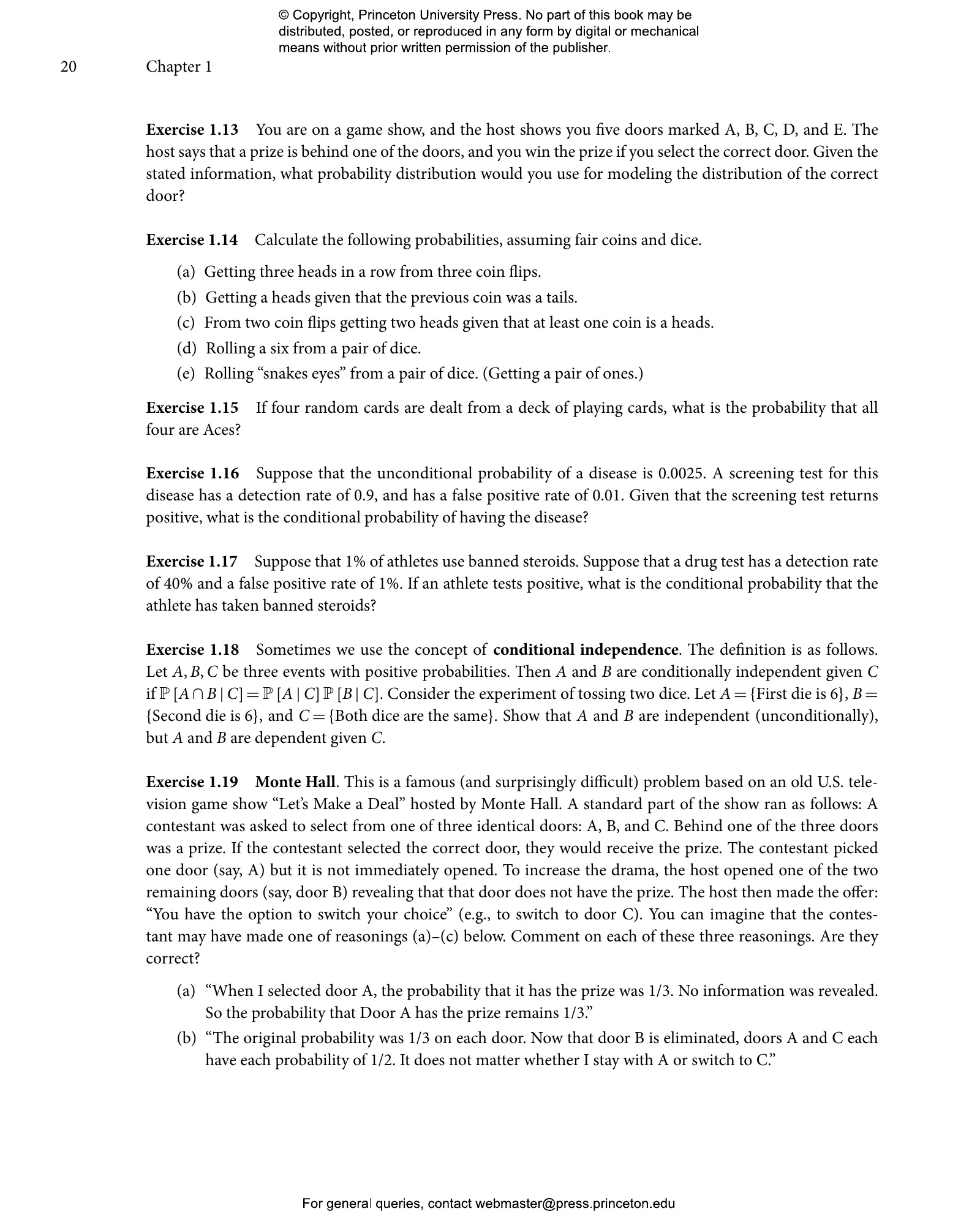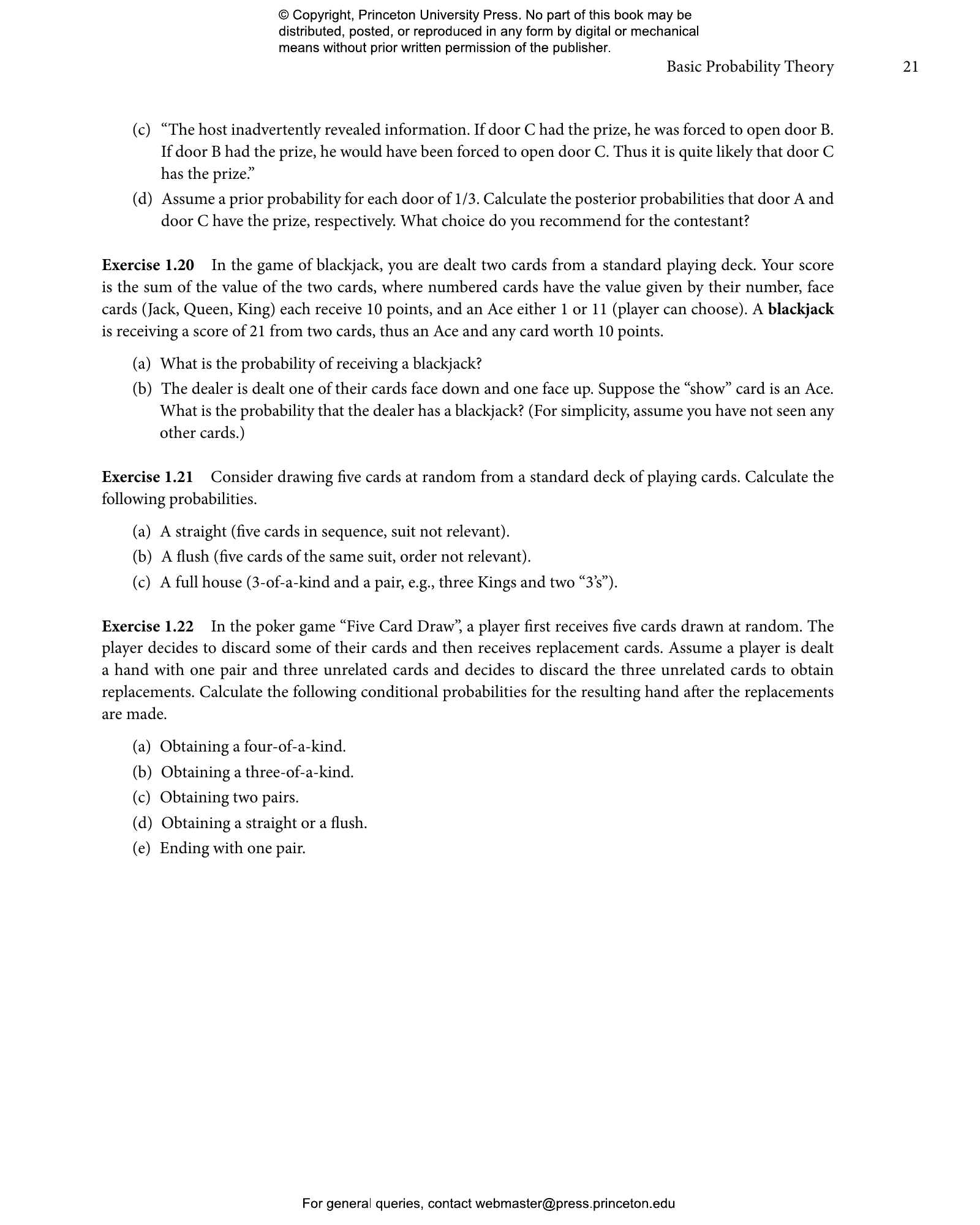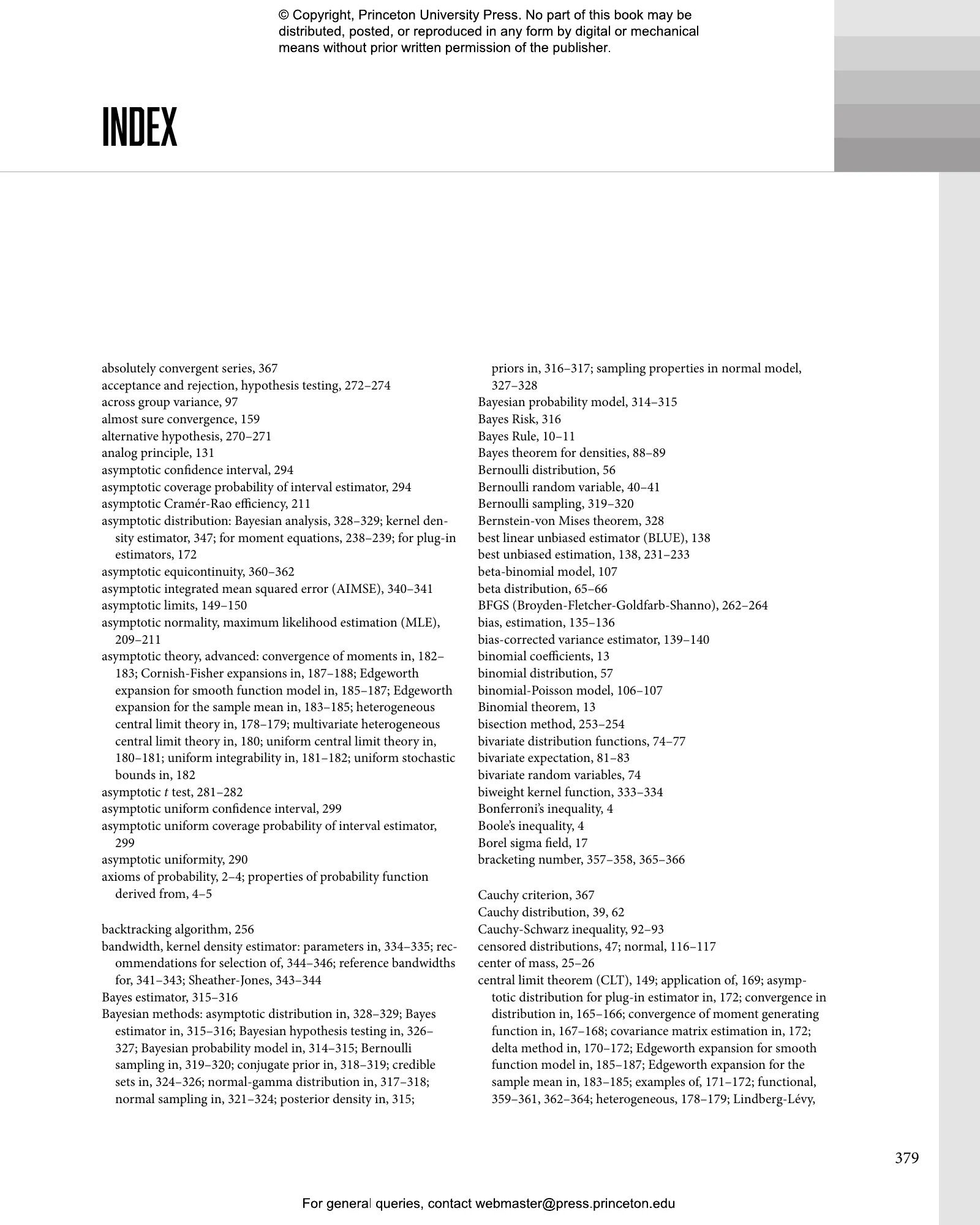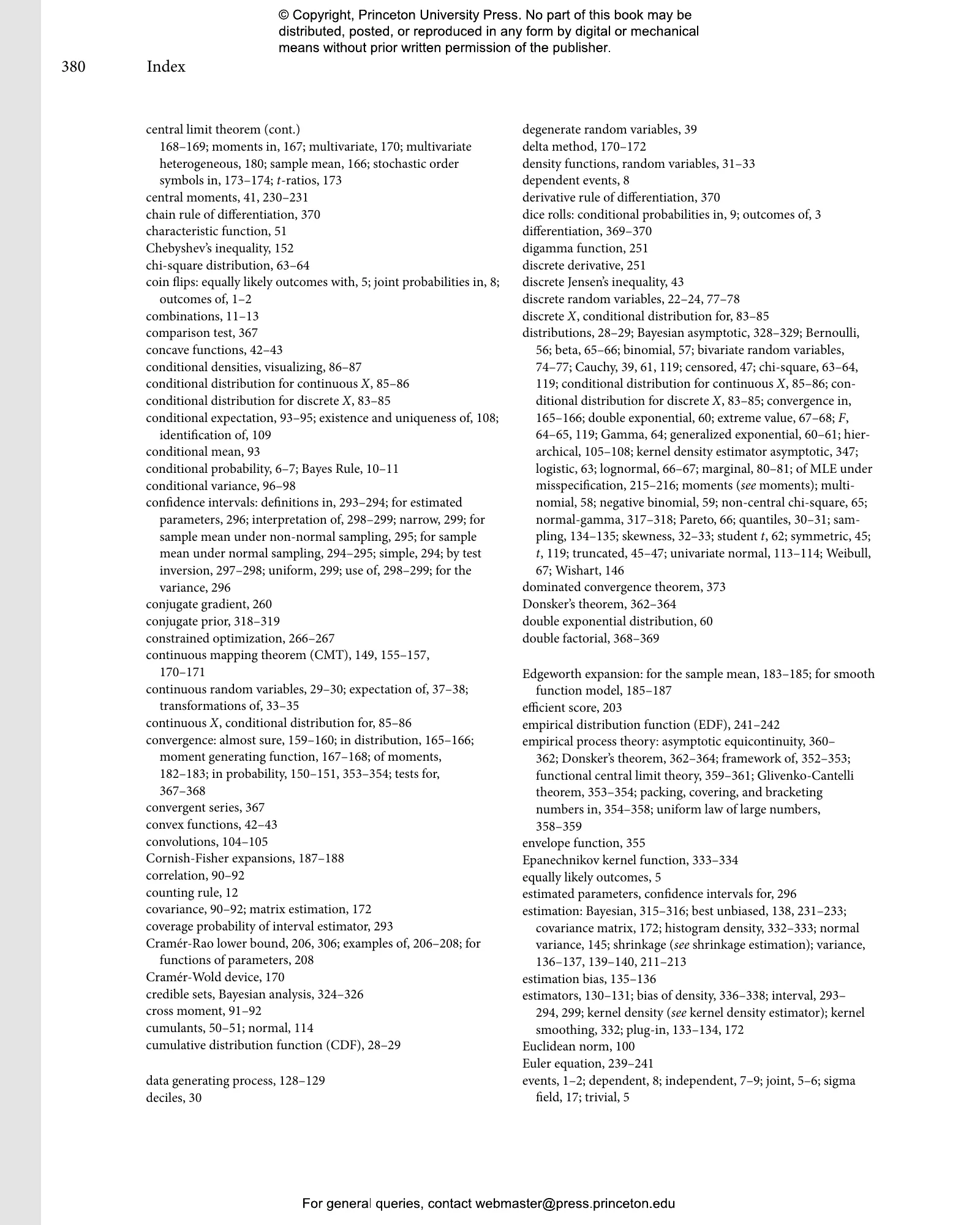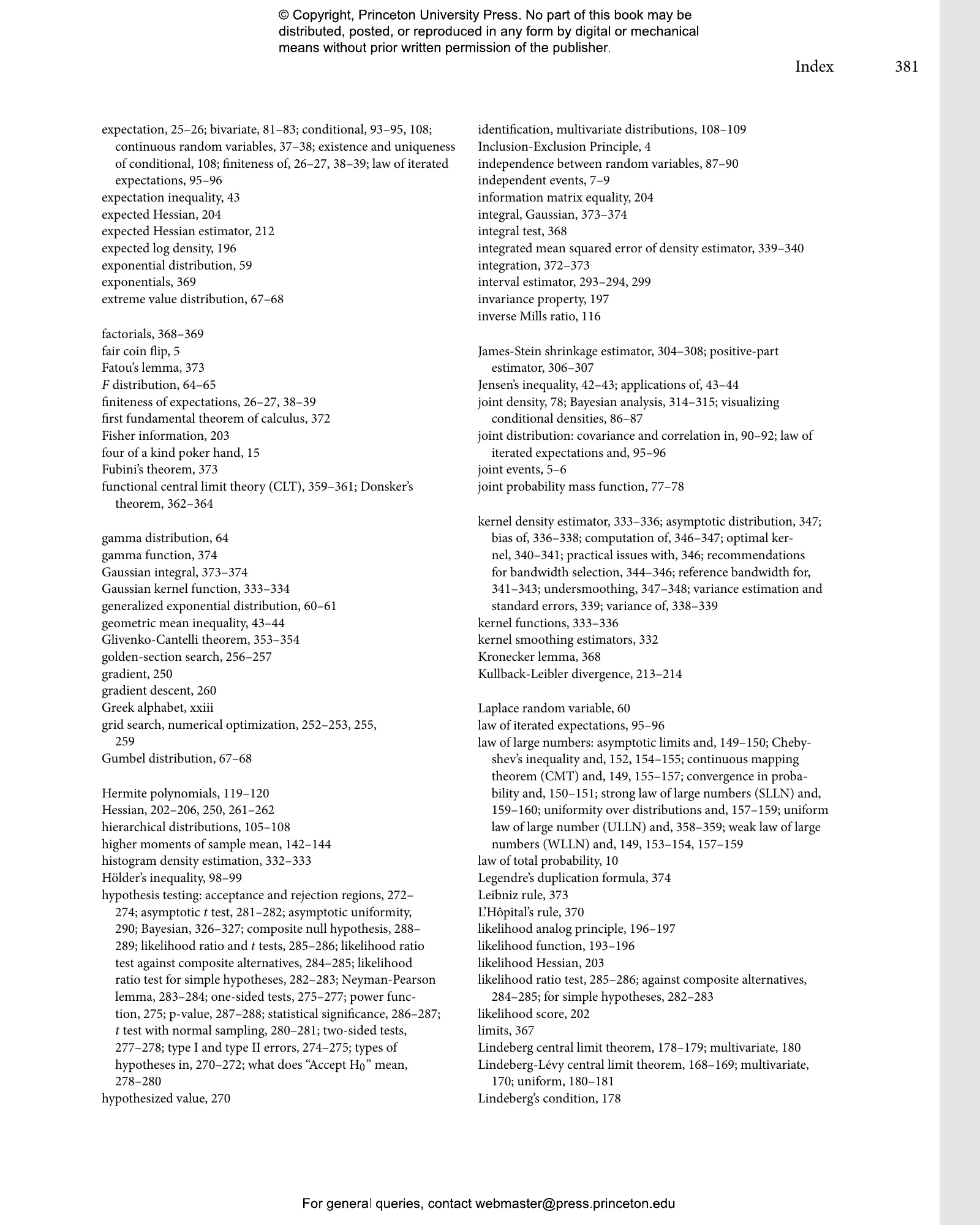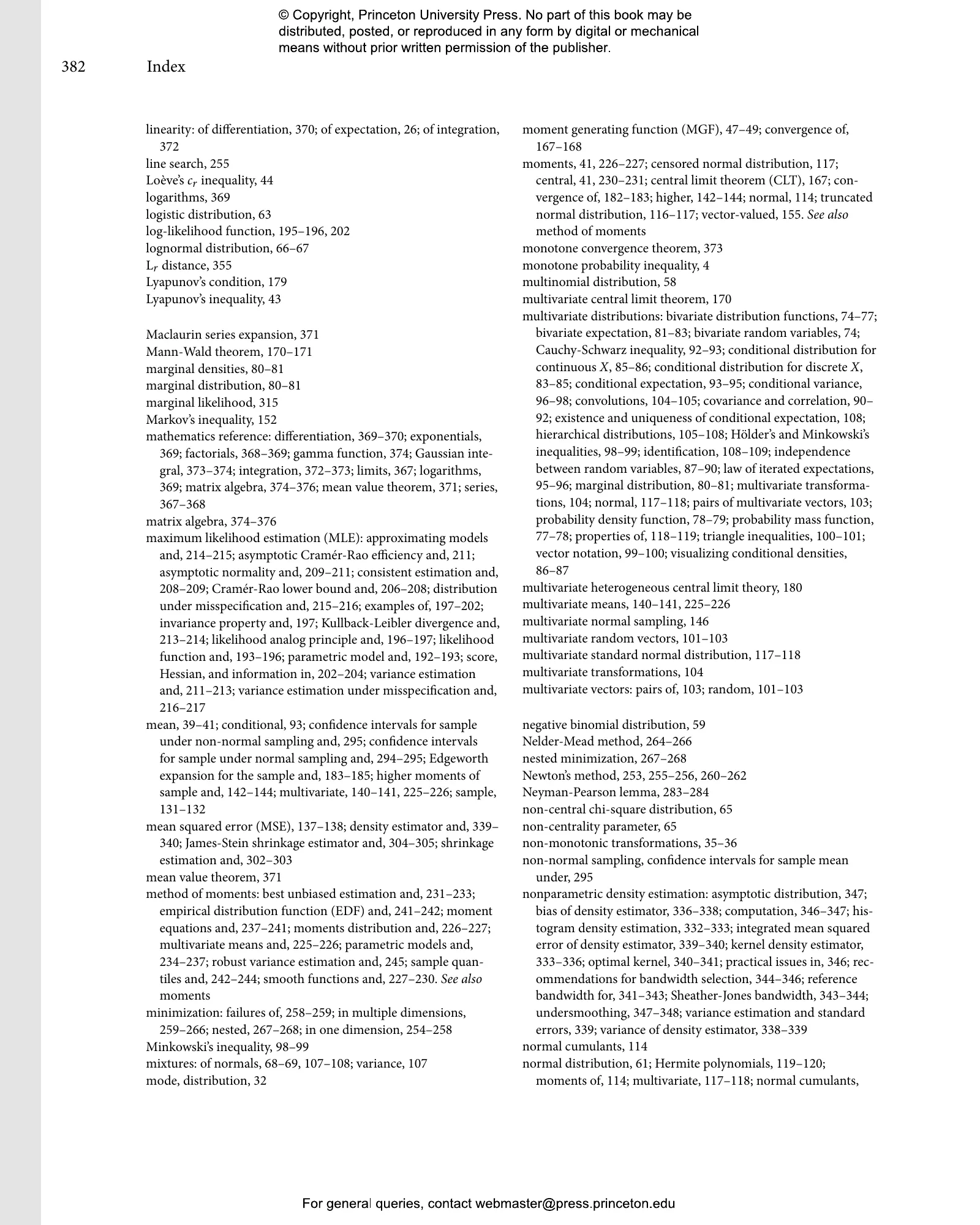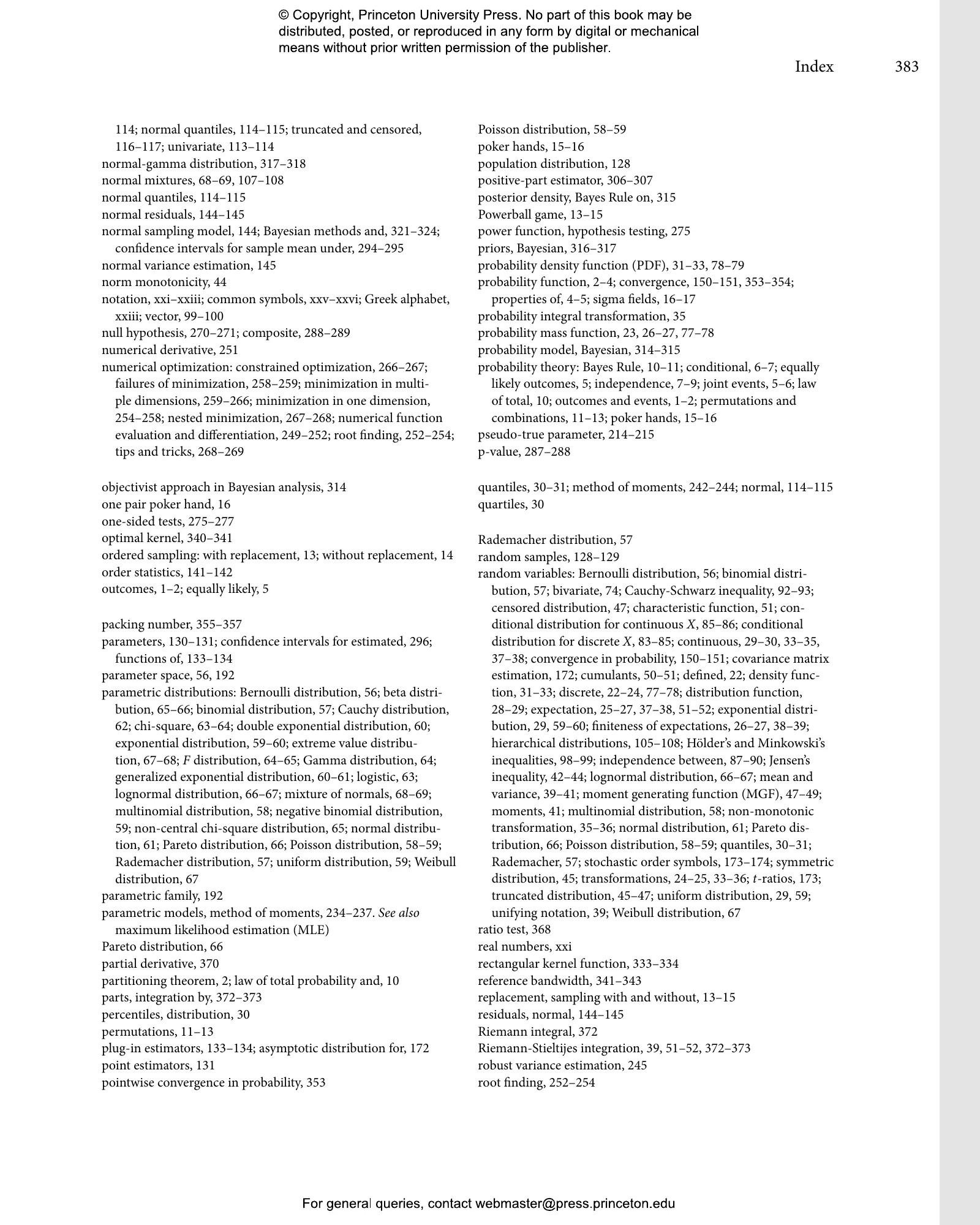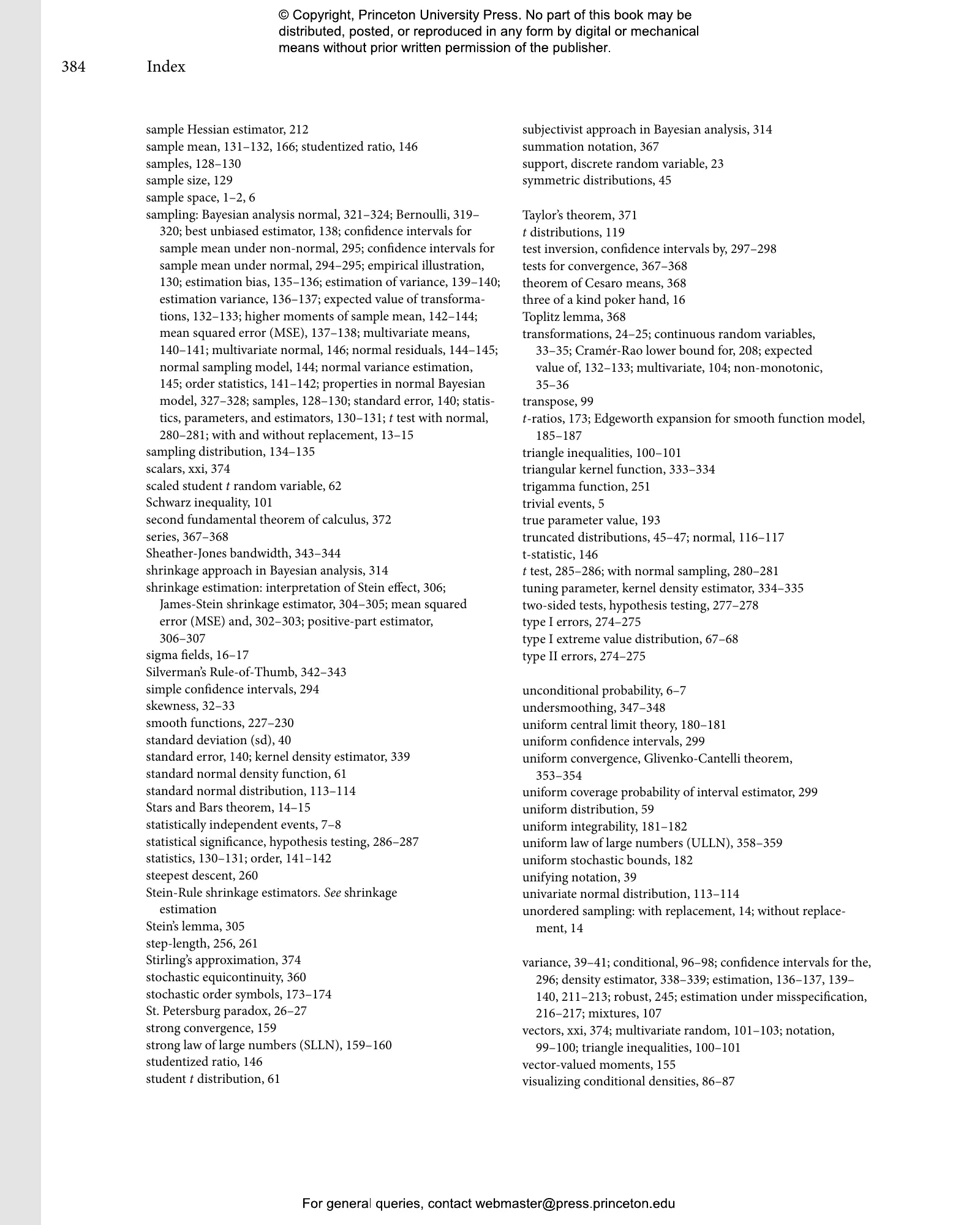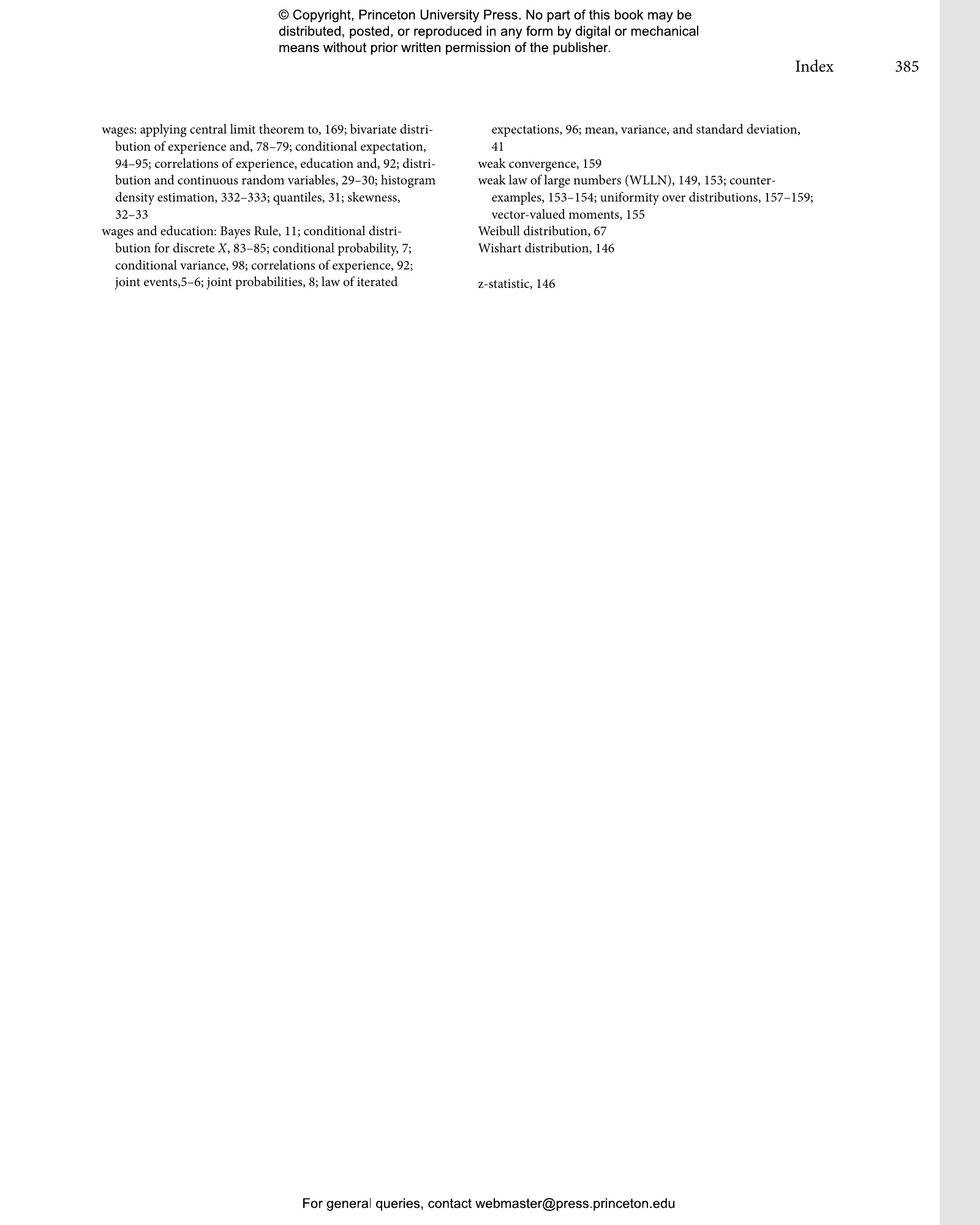#### Probability and Statistics for Economists

Bruce Hansen

##### Before you purchase audiobooks and ebooks

Please note that audiobooks and ebooks purchased from this site must be accessed on the Princeton University Press app. After you make your purchase, you will receive an email with instructions on how to download the app.

# Probability and Statistics for Economists

A comprehensive and up-to-date introduction to the mathematics that all economics students need to know## Hardcover

Price:
\$70.00/£58.00
ISBN:
Published (US):
Aug 23, 2022
Published (UK):
Oct 18, 2022
2022
Pages:
416
Size:
8 x 10 in.
Illus:
111 b/w illus. 6 tables.

Probability theory is the quantitative language used to handle uncertainty and is the foundation of modern statistics. Probability and Statistics for Economists provides graduate and PhD students with an essential introduction to mathematical probability and statistical theory, which are the basis of the methods used in econometrics. This incisive textbook teaches fundamental concepts, emphasizes modern, real-world applications, and gives students an intuitive understanding of the mathematics that every economist needs to know.

• Covers probability and statistics with mathematical rigor while emphasizing intuitive explanations that are accessible to economics students of all backgrounds
• Discusses random variables, parametric and multivariate distributions, sampling, the law of large numbers, central limit theory, maximum likelihood estimation, numerical optimization, hypothesis testing, and more
• Features hundreds of exercises that enable students to learn by doing
• Includes an in-depth appendix summarizing important mathematical results as well as a wealth of real-world examples
• Can serve as a core textbook for a first-semester PhD course in econometrics and as a companion book to Bruce E. Hansen’s Econometrics
• Also an invaluable reference for researchers and practitioners